Скачать презентацию Market Risk Modelling By A V Vedpuriswar July

• Количество слайдов: 69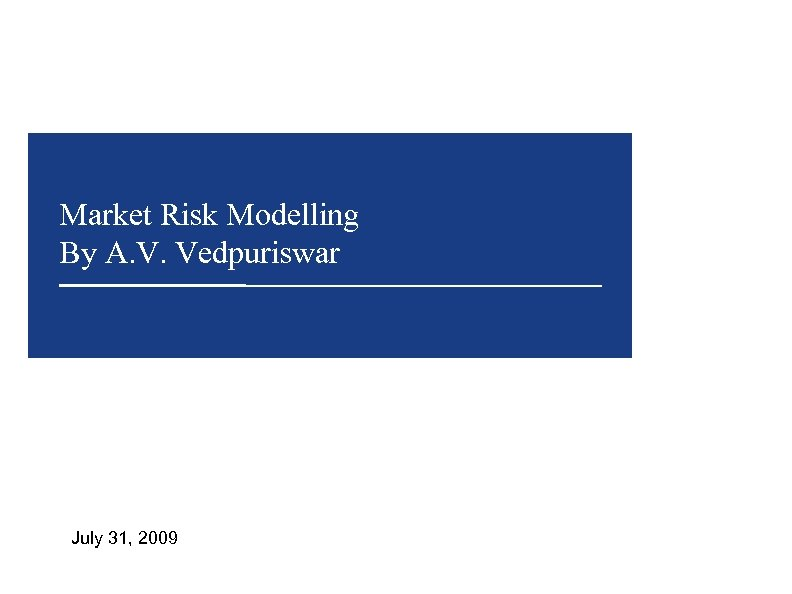Market Risk Modelling By A. V. Vedpuriswar July 31, 2009Volatility 1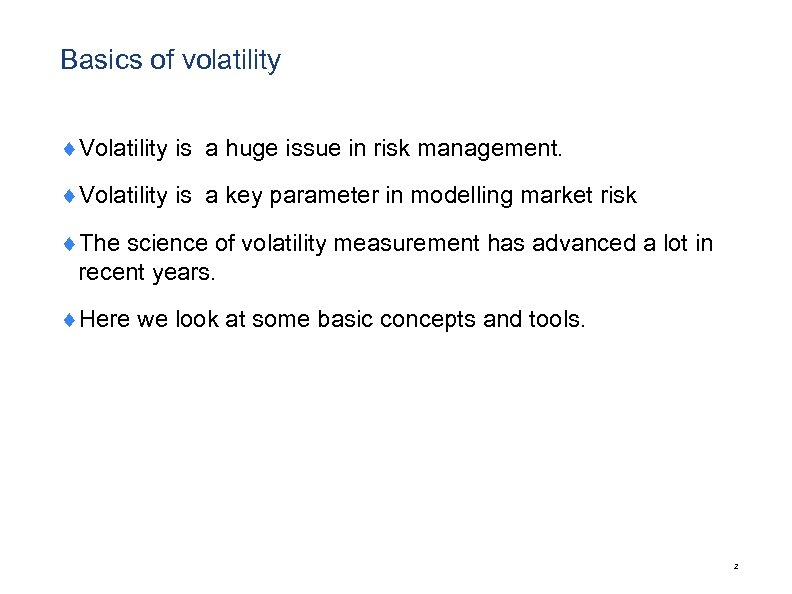Basics of volatility ¨Volatility is a huge issue in risk management. ¨Volatility is a key parameter in modelling market risk ¨The science of volatility measurement has advanced a lot in recent years. ¨Here we look at some basic concepts and tools. 2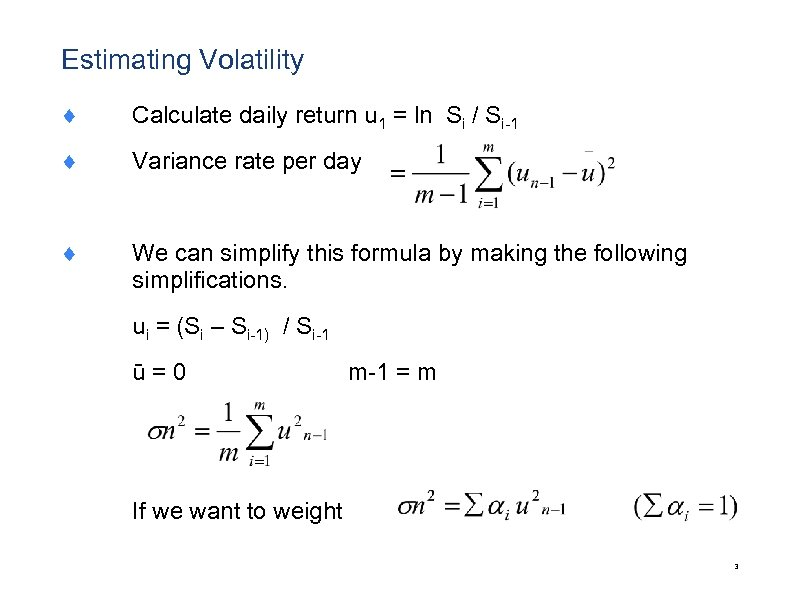Estimating Volatility ¨ Calculate daily return u 1 = ln Si / Si-1 ¨ Variance rate per day ¨ We can simplify this formula by making the following simplifications. ui = (Si – Si-1) / Si-1 ū = 0 m-1 = m If we want to weight 3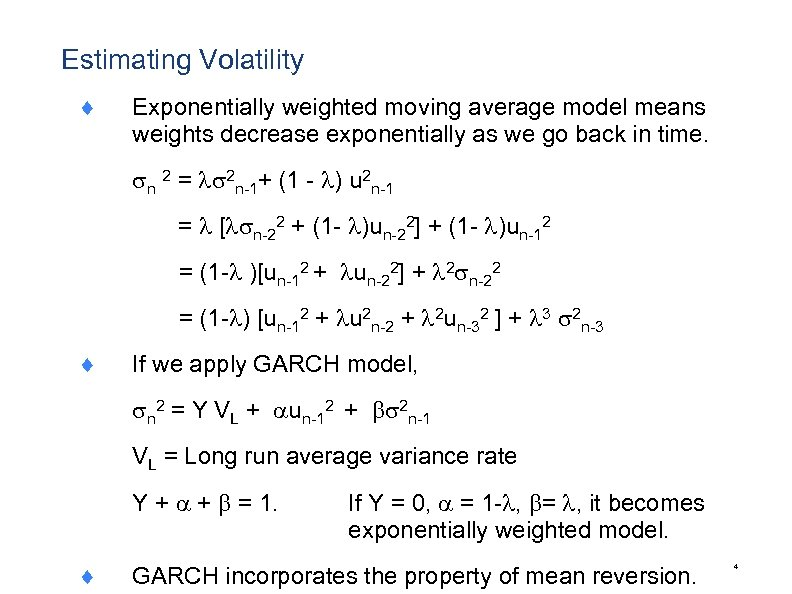Estimating Volatility ¨ Exponentially weighted moving average model means weights decrease exponentially as we go back in time. n 2 = 2 n-1+ (1 - ) u 2 n-1 = [ n-22 + (1 - )un-22] + (1 - )un-12 = (1 - )[un-12 + un-22] + 2 n-22 = (1 - ) [un-12 + u 2 n-2 + 2 un-32 ] + 3 2 n-3 ¨ If we apply GARCH model, n 2 = Y VL + un-12 + 2 n-1 VL = Long run average variance rate Y + + = 1. ¨ If Y = 0, = 1 - , = , it becomes exponentially weighted model. GARCH incorporates the property of mean reversion. 4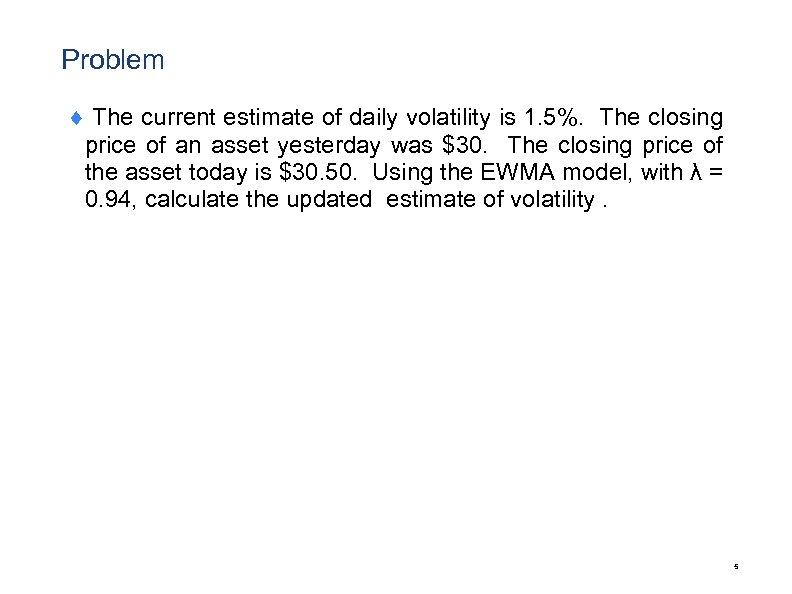Problem ¨ The current estimate of daily volatility is 1. 5%. The closing price of an asset yesterday was \$30. The closing price of the asset today is \$30. 50. Using the EWMA model, with λ = 0. 94, calculate the updated estimate of volatility. 5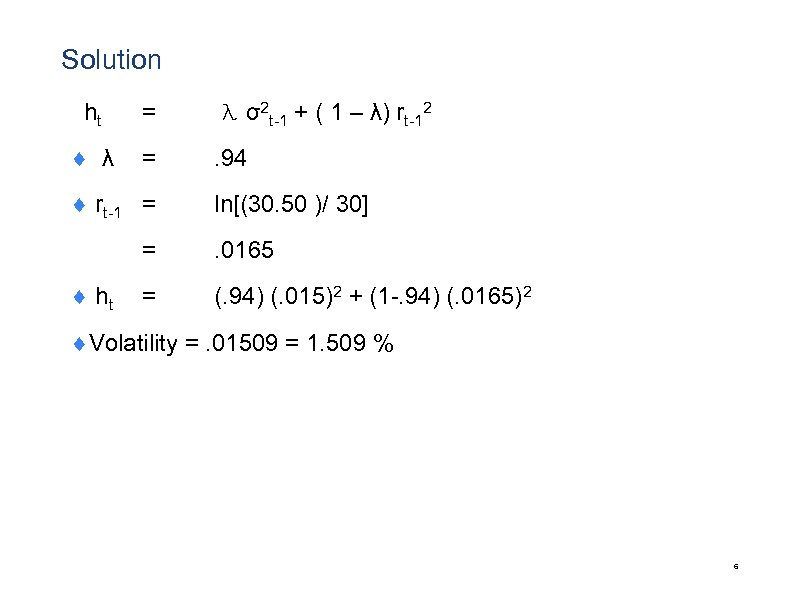Solution ht = λ σ2 t-1 + ( 1 – λ) rt-12 ¨ λ = . 94 ¨ rt-1 = ln[(30. 50 )/ 30] = ¨ ht . 0165 = (. 94) (. 015)2 + (1 -. 94) (. 0165)2 ¨Volatility =. 01509 = 1. 509 % 6Greeks 7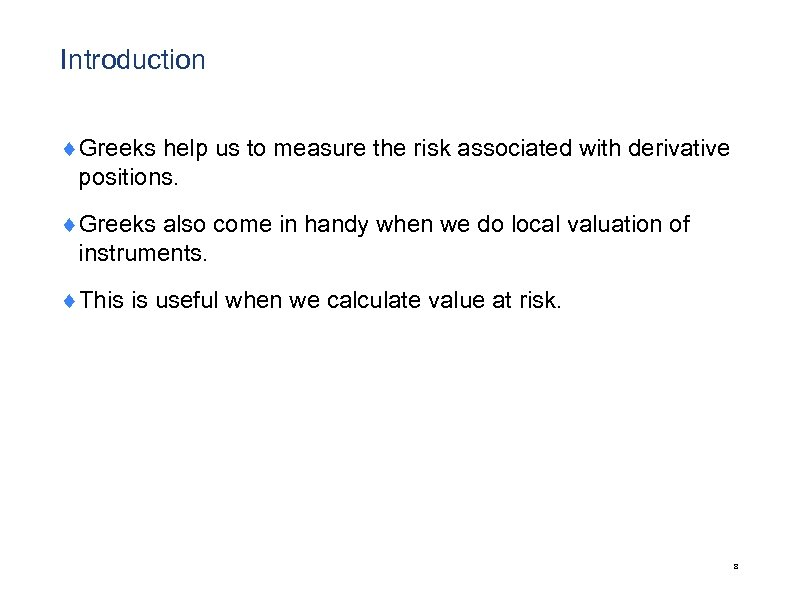Introduction ¨Greeks help us to measure the risk associated with derivative positions. ¨Greeks also come in handy when we do local valuation of instruments. ¨This is useful when we calculate value at risk. 8Delta ¨ 9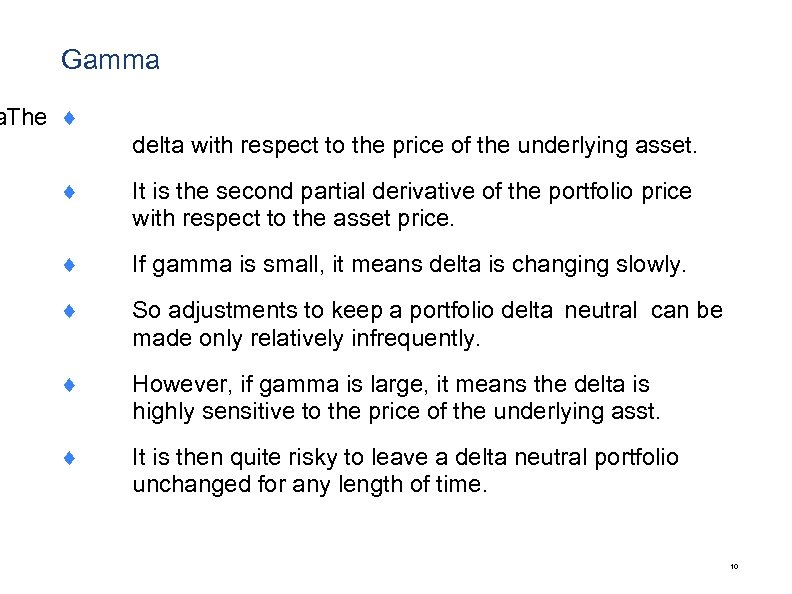Gamma a The ¨ delta with respect to the price of the underlying asset. ¨ It is the second partial derivative of the portfolio price with respect to the asset price. ¨ If gamma is small, it means delta is changing slowly. ¨ So adjustments to keep a portfolio delta neutral can be made only relatively infrequently. ¨ However, if gamma is large, it means the delta is highly sensitive to the price of the underlying asst. ¨ It is then quite risky to leave a delta neutral portfolio unchanged for any length of time. 10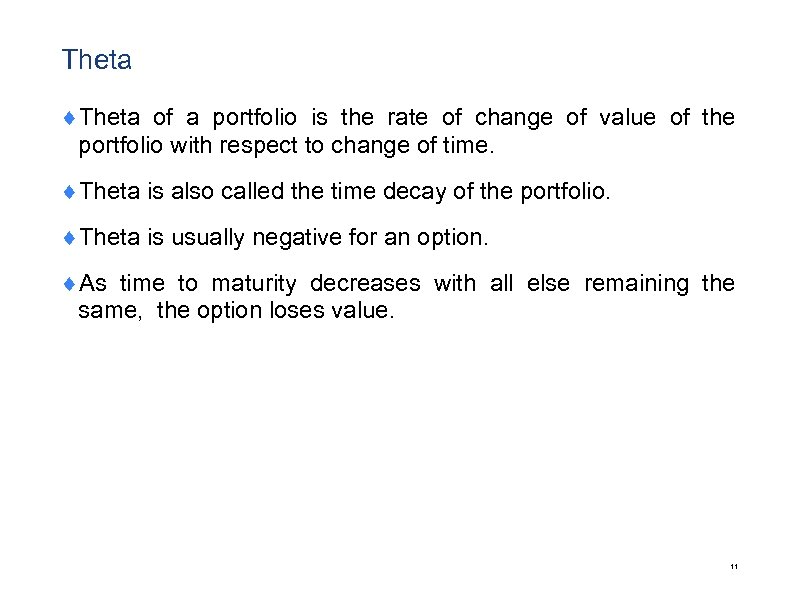Theta ¨Theta of a portfolio is the rate of change of value of the portfolio with respect to change of time. ¨Theta is also called the time decay of the portfolio. ¨Theta is usually negative for an option. ¨As time to maturity decreases with all else remaining the same, the option loses value. 11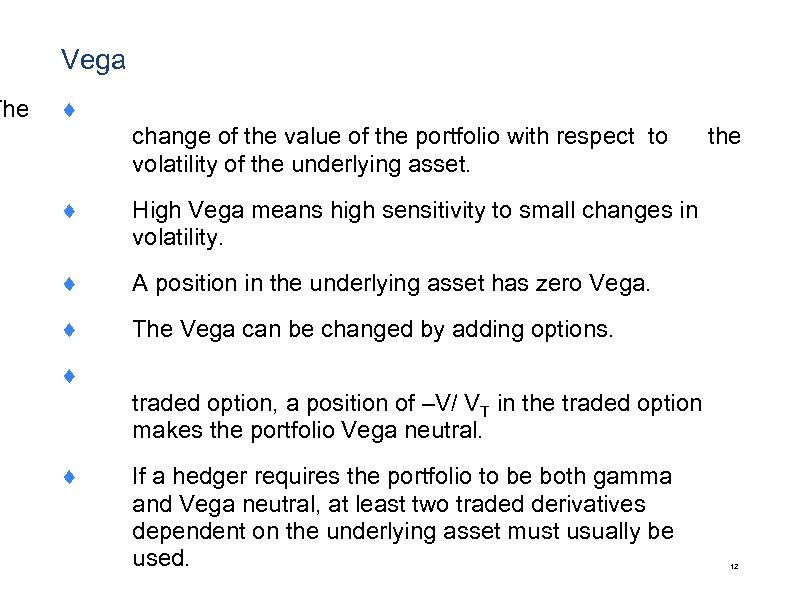The Vega ¨ change of the value of the portfolio with respect to volatility of the underlying asset. ¨ High Vega means high sensitivity to small changes in volatility. ¨ the A position in the underlying asset has zero Vega. ¨ The Vega can be changed by adding options. ¨ traded option, a position of –V/ VT in the traded option makes the portfolio Vega neutral. ¨ If a hedger requires the portfolio to be both gamma and Vega neutral, at least two traded derivatives dependent on the underlying asset must usually be used. 12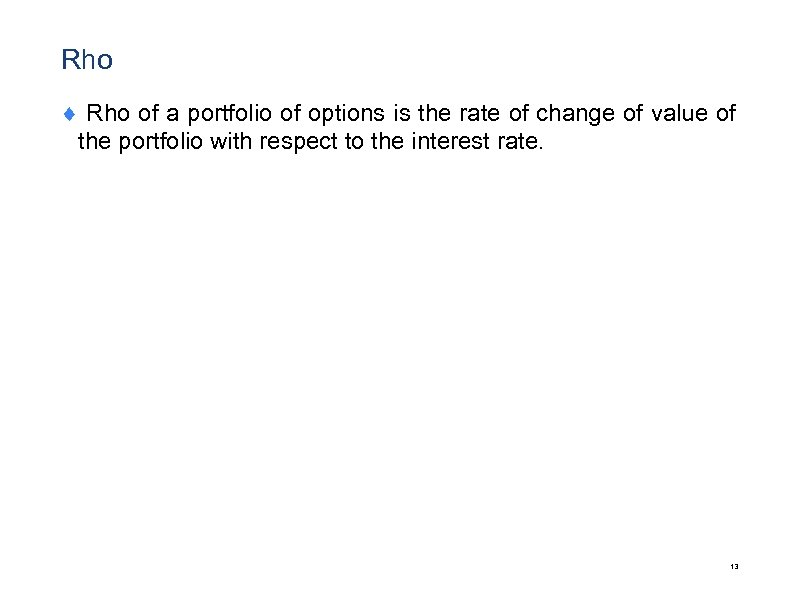Rho ¨ Rho of a portfolio of options is the rate of change of value of the portfolio with respect to the interest rate. 13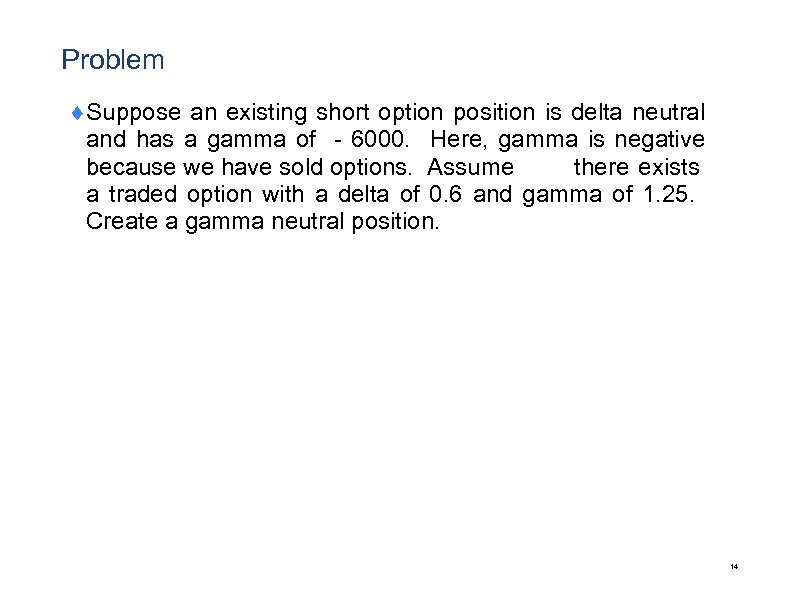Problem ¨Suppose an existing short option position is delta neutral and has a gamma of －6000. Here, gamma is negative because we have sold options. Assume there exists a traded option with a delta of 0. 6 and gamma of 1. 25. Create a gamma neutral position. 14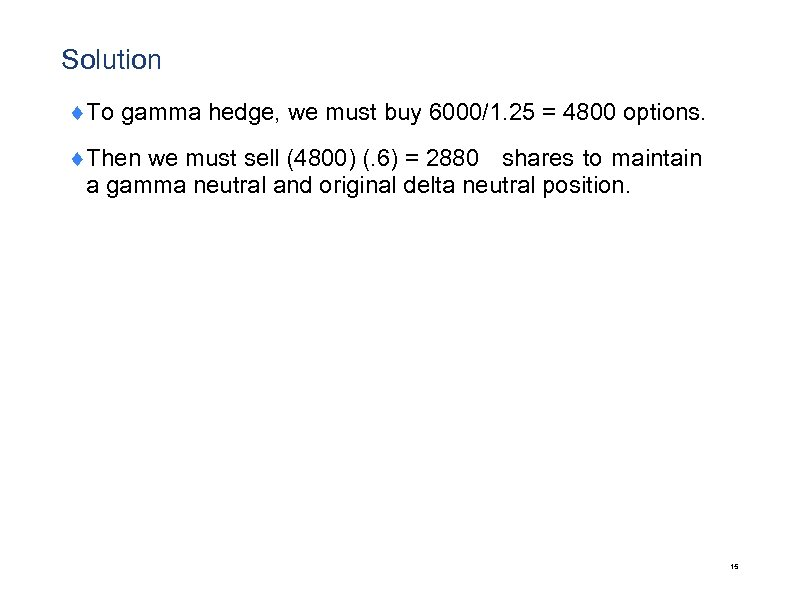Solution ¨To gamma hedge, we must buy 6000/1. 25 = 4800 options. ¨Then we must sell (4800) (. 6) = 2880 shares to maintain a gamma neutral and original delta neutral position. 15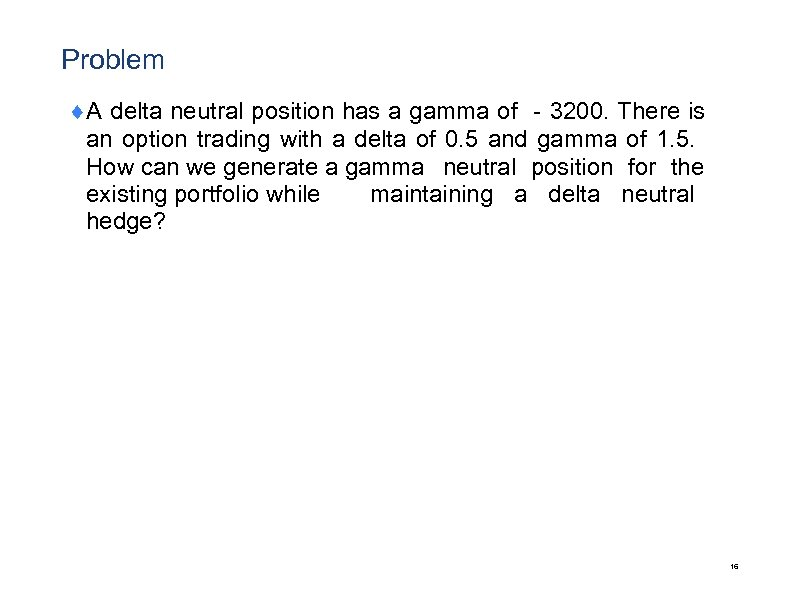Problem ¨A delta neutral position has a gamma of －3200. There is an option trading with a delta of 0. 5 and gamma of 1. 5. How can we generate a gamma neutral position for the existing portfolio while maintaining a delta neutral hedge? 16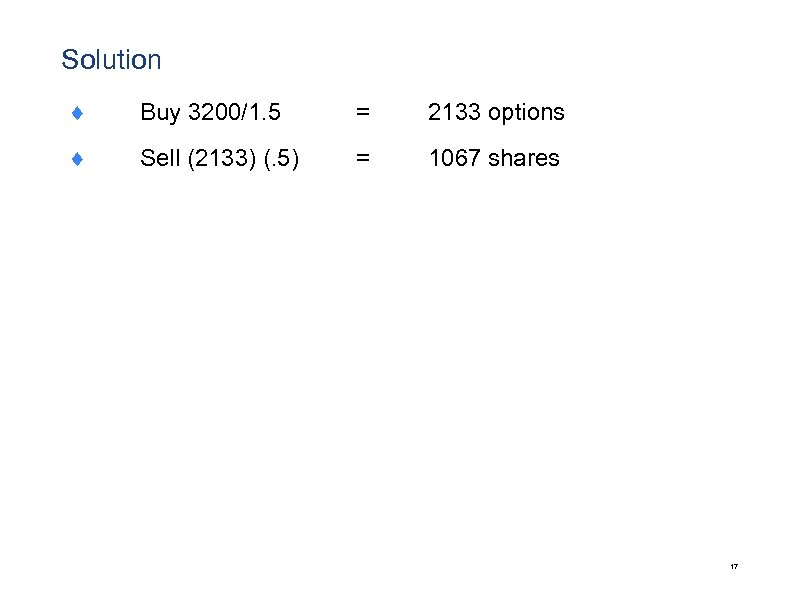Solution ¨ Buy 3200/1. 5 = 2133 options ¨ Sell (2133) (. 5) = 1067 shares 17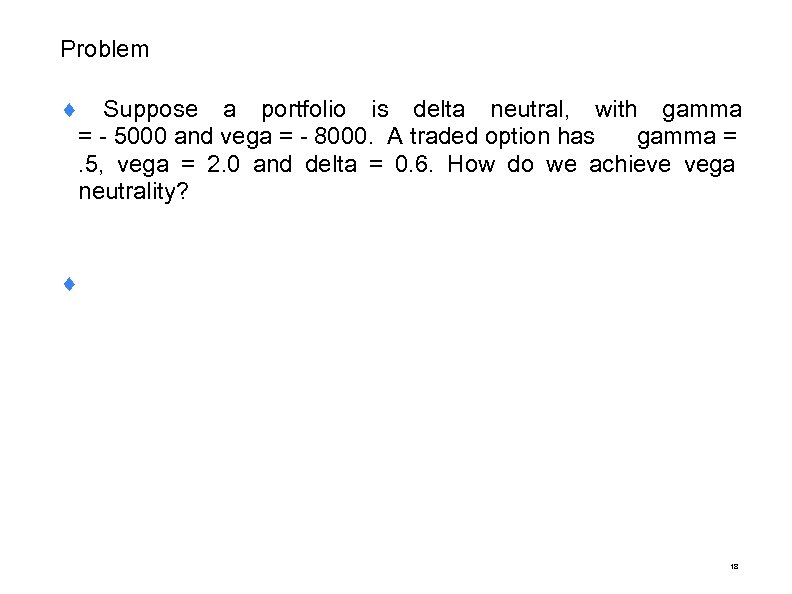Problem ¨ Suppose a portfolio is delta neutral, with gamma = - 5000 and vega = - 8000. A traded option has gamma = . 5, vega = 2. 0 and delta = 0. 6. How do we achieve vega neutrality? ¨ 18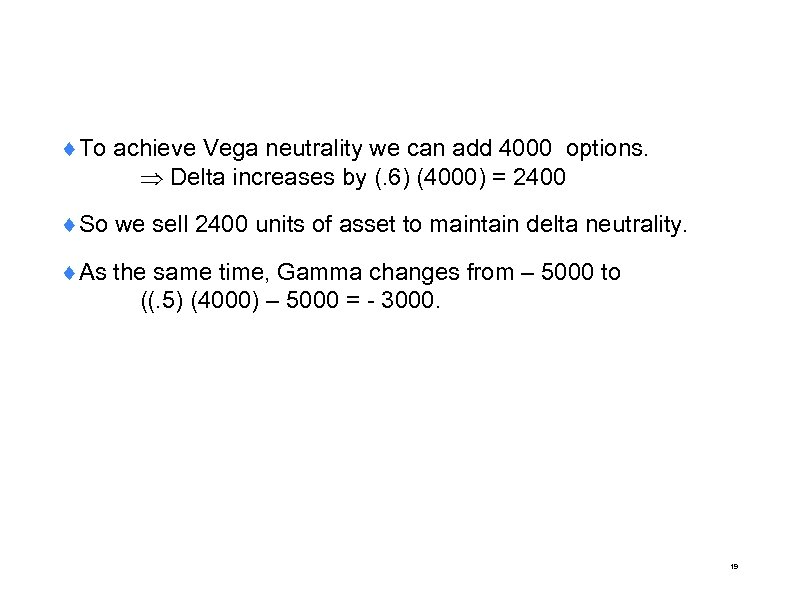¨To achieve Vega neutrality we can add 4000 options. Delta increases by (. 6) (4000) = 2400 ¨So we sell 2400 units of asset to maintain delta neutrality. ¨As the same time, Gamma changes from – 5000 to ((. 5) (4000) – 5000 = - 3000. 19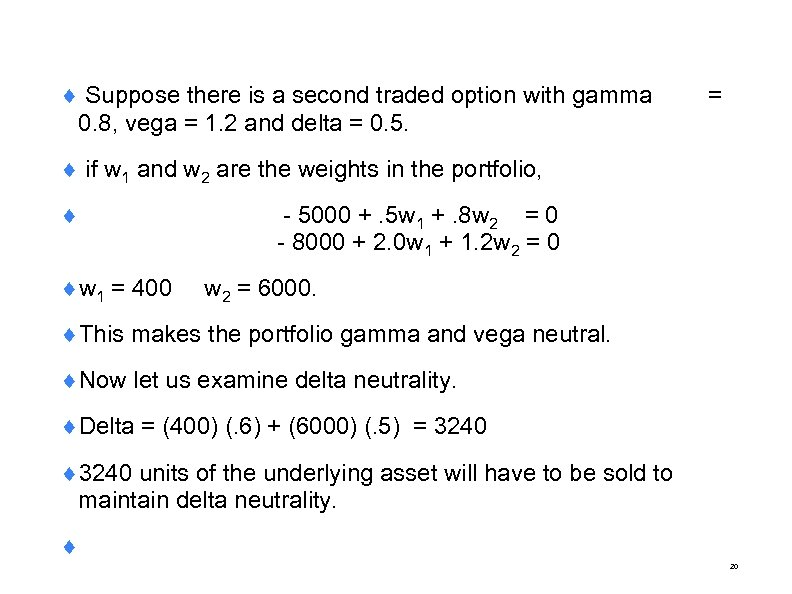¨ Suppose there is a second traded option with gamma 0. 8, vega = 1. 2 and delta = 0. 5. = ¨ if w 1 and w 2 are the weights in the portfolio, ¨ - 5000 +. 5 w 1 +. 8 w 2 = 0 - 8000 + 2. 0 w 1 + 1. 2 w 2 = 0 ¨w 1 = 400 w 2 = 6000. ¨This makes the portfolio gamma and vega neutral. ¨Now let us examine delta neutrality. ¨Delta = (400) (. 6) + (6000) (. 5) = 3240 ¨ 3240 units of the underlying asset will have to be sold to maintain delta neutrality. ¨ 20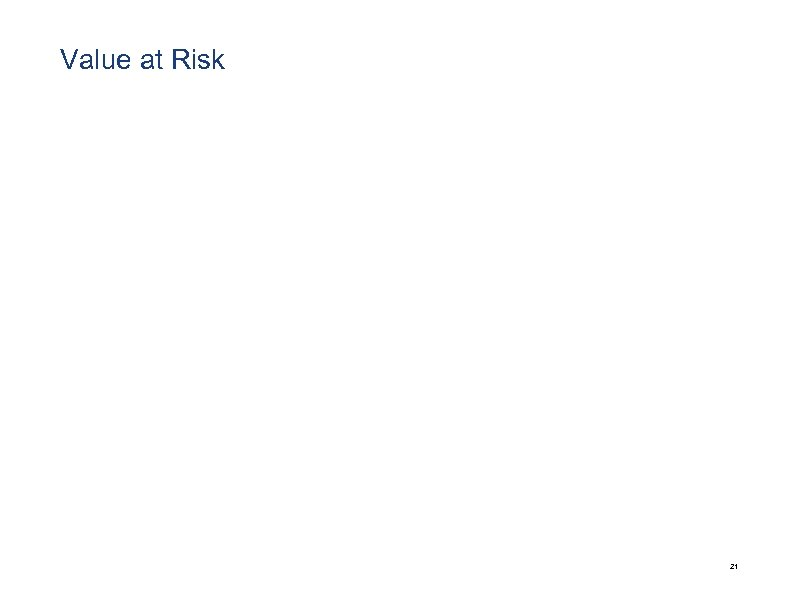Value at Risk 21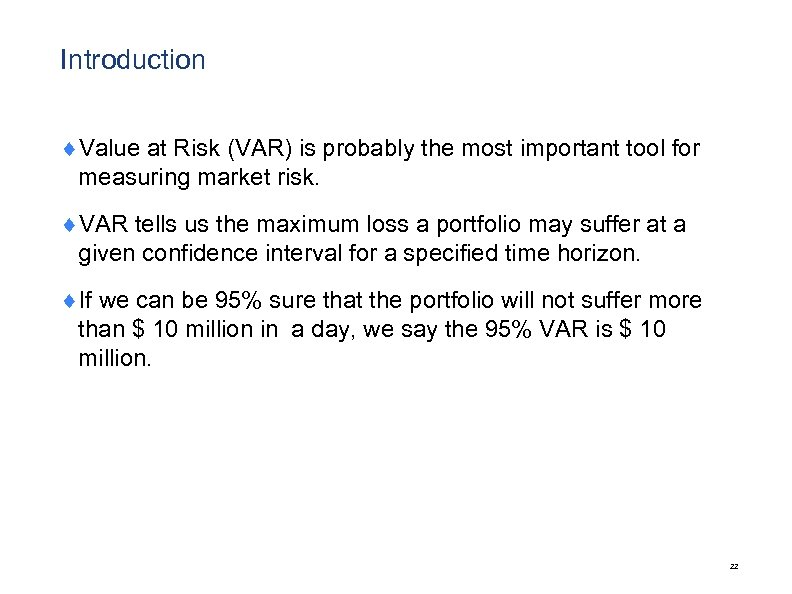Introduction ¨Value at Risk (VAR) is probably the most important tool for measuring market risk. ¨VAR tells us the maximum loss a portfolio may suffer at a given confidence interval for a specified time horizon. ¨If we can be 95% sure that the portfolio will not suffer more than \$ 10 million in a day, we say the 95% VAR is \$ 10 million. 22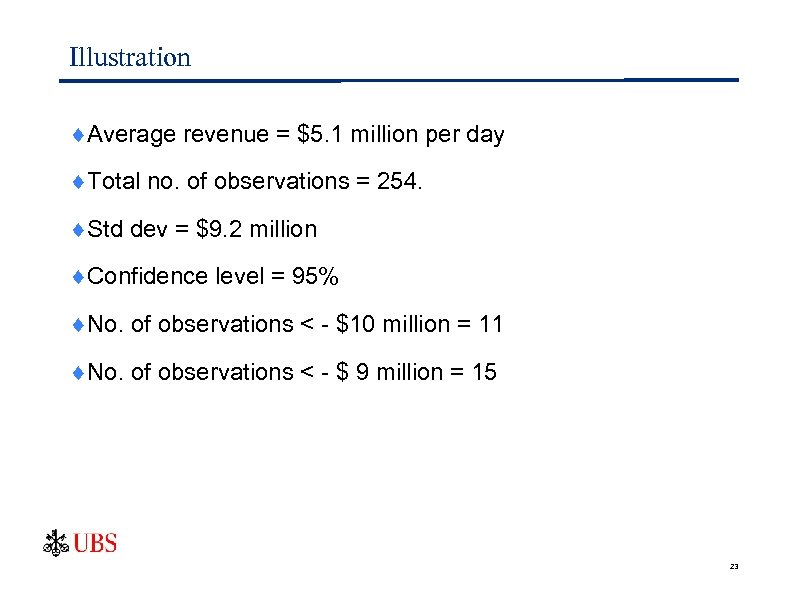Illustration ¨Average revenue = \$5. 1 million per day ¨Total no. of observations = 254. ¨Std dev = \$9. 2 million ¨Confidence level = 95% ¨No. of observations < - \$10 million = 11 ¨No. of observations < - \$ 9 million = 15 23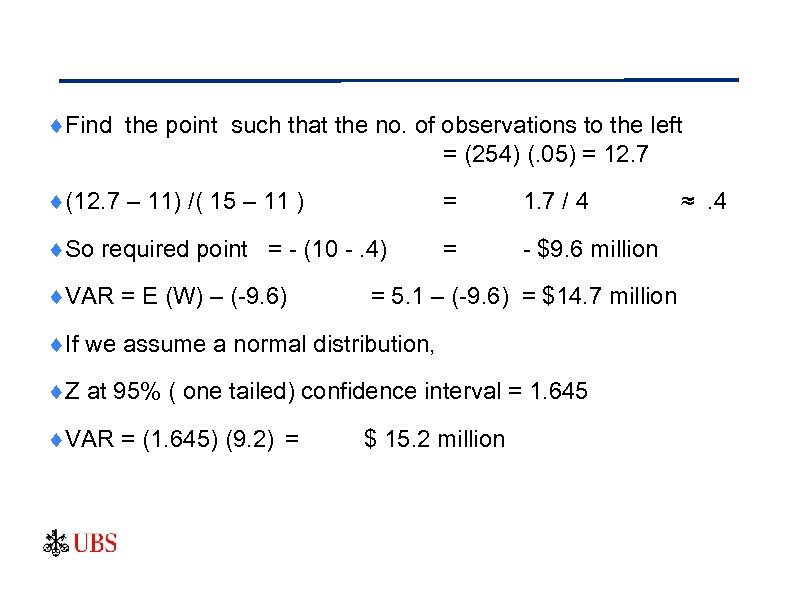¨Find the point such that the no. of observations to the left = (254) (. 05) = 12. 7 ¨(12. 7 – 11) /( 15 – 11 ) = 1. 7 / 4 ¨So required point = - (10 -. 4) = - \$9. 6 million ¨VAR = E (W) – (-9. 6) = 5. 1 – (-9. 6) = \$14. 7 million ¨If we assume a normal distribution, ¨Z at 95% ( one tailed) confidence interval = 1. 645 ¨VAR = (1. 645) (9. 2) = \$ 15. 2 million ≈ . 4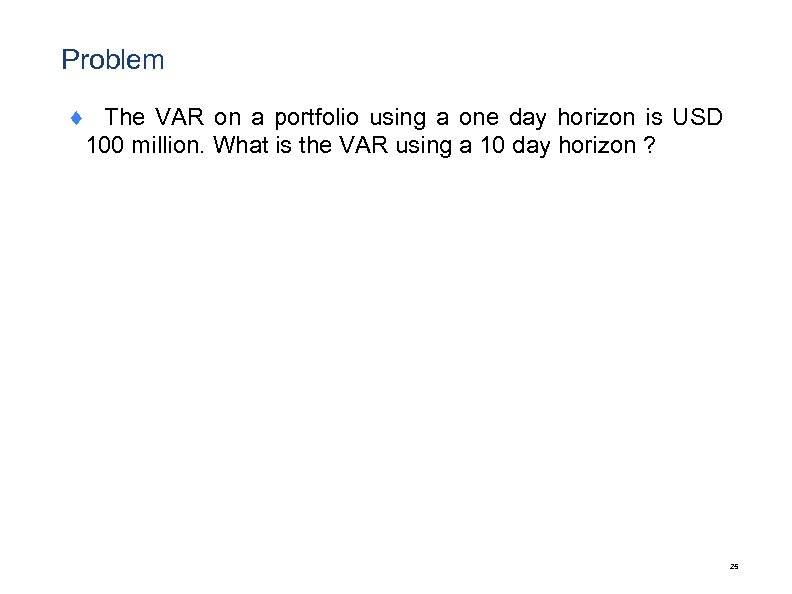Problem ¨ The VAR on a portfolio using a one day horizon is USD 100 million. What is the VAR using a 10 day horizon ? 25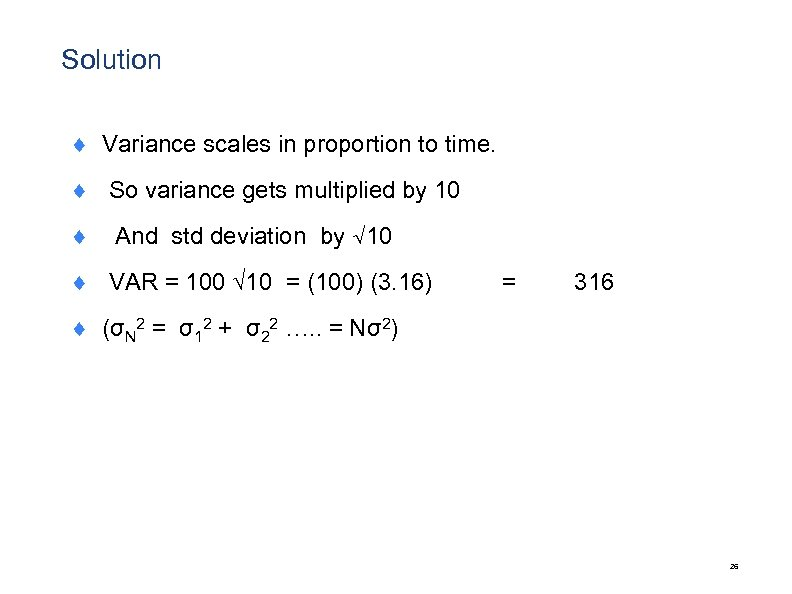Solution ¨ Variance scales in proportion to time. ¨ So variance gets multiplied by 10 ¨ And std deviation by √ 10 ¨ VAR = 100 √ 10 = (100) (3. 16) = 316 ¨ (σN 2 = σ12 + σ22 …. . = Nσ2) 26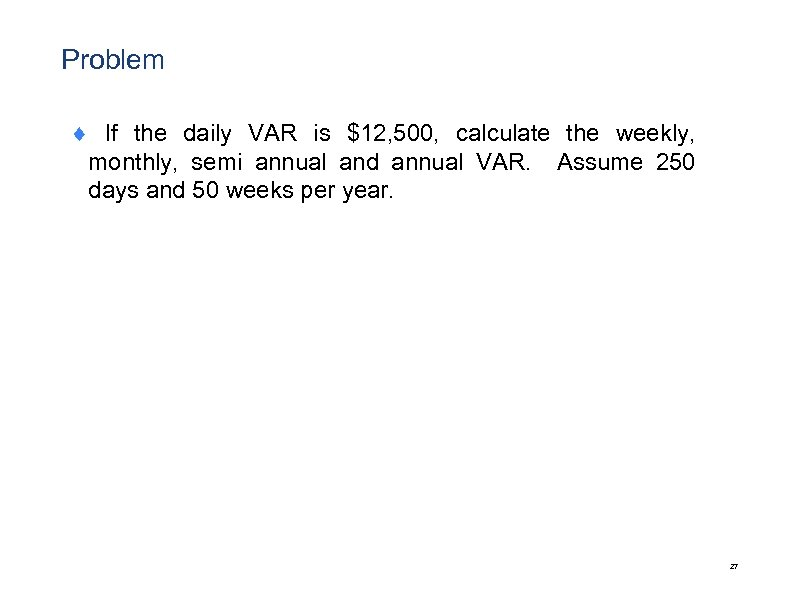Problem ¨ If the daily VAR is \$12, 500, calculate the weekly, monthly, semi annual and annual VAR. Assume 250 days and 50 weeks per year. 27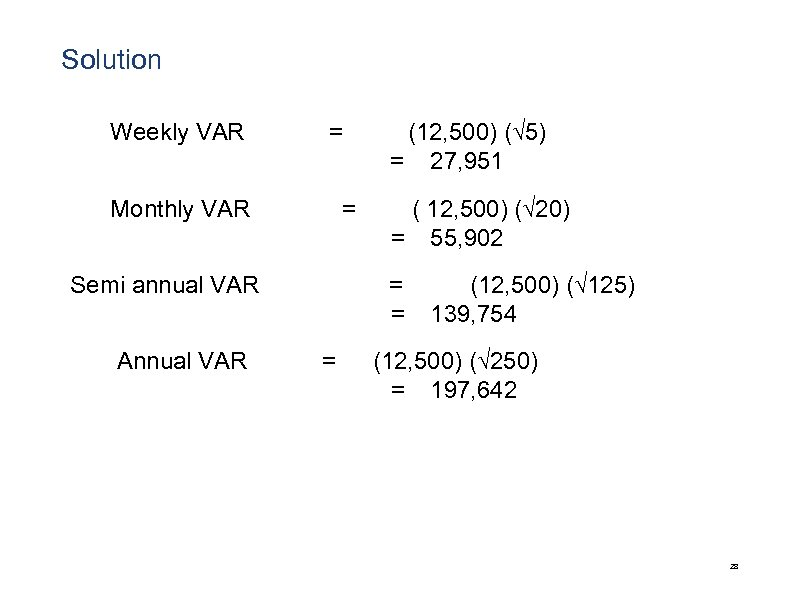Solution Weekly VAR ＝ (12, 500) (√ 5) ＝ 27, 951 Monthly VAR ＝ ( 12, 500) (√ 20) ＝ 55, 902 Semi annual VAR ＝ (12, 500) (√ 125) ＝ 139, 754 Annual VAR ＝ (12, 500) (√ 250) ＝ 197, 642 28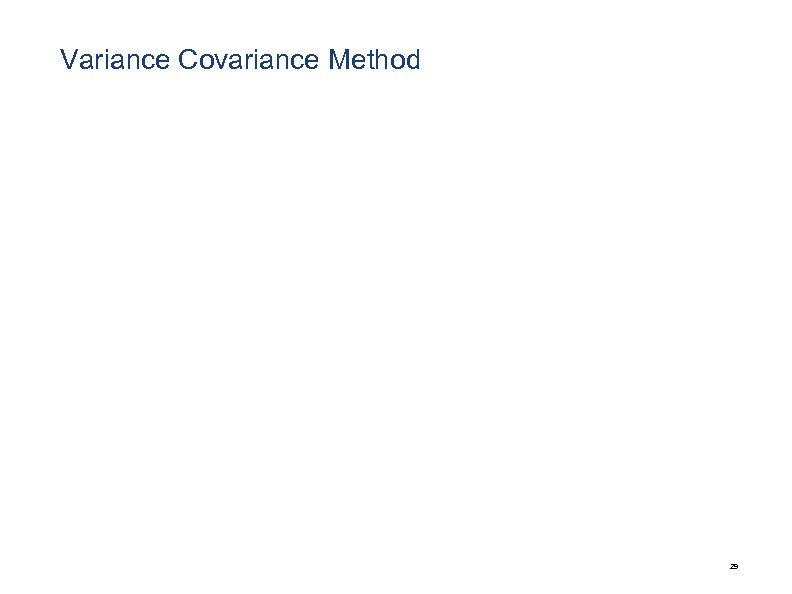Variance Covariance Method 29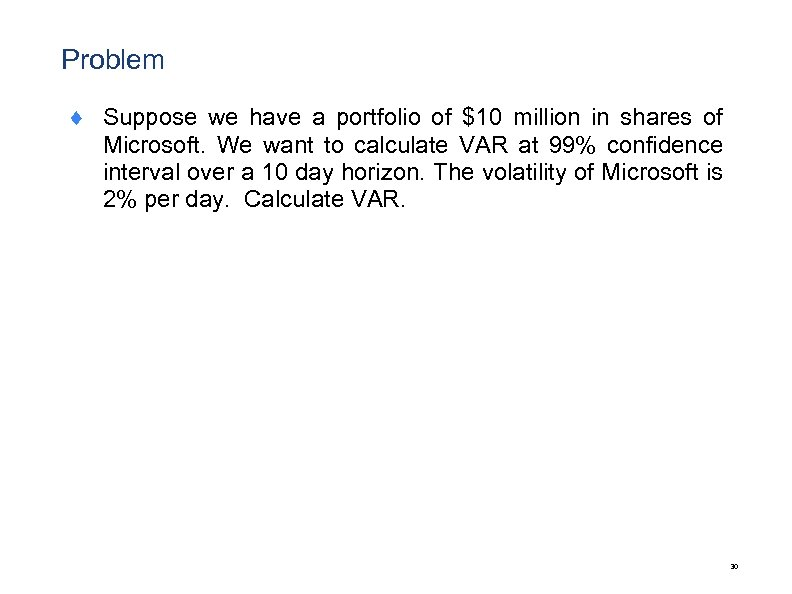Problem ¨ Suppose we have a portfolio of \$10 million in shares of Microsoft. We want to calculate VAR at 99% confidence interval over a 10 day horizon. The volatility of Microsoft is 2% per day. Calculate VAR. 30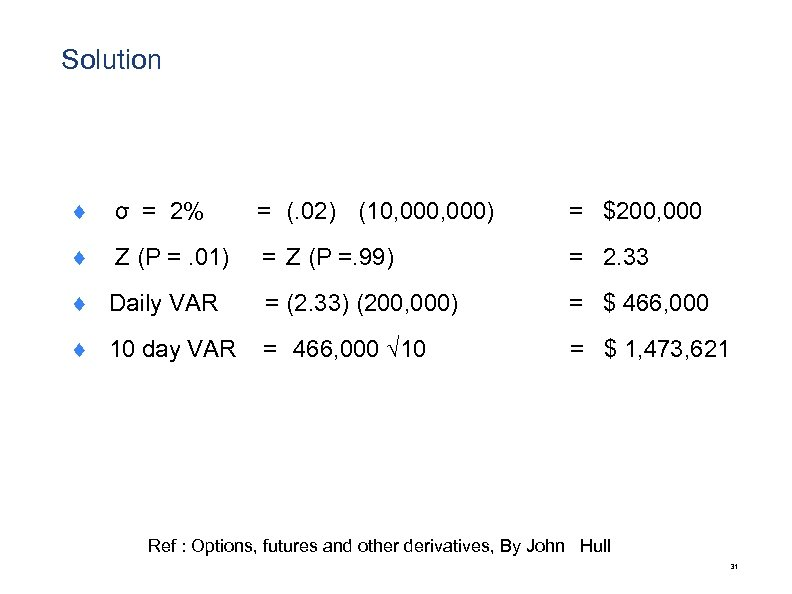Solution ¨ σ = 2% = (. 02) (10, 000) = \$200, 000 ¨ Z (P =. 01) = Z (P =. 99) = 2. 33 ¨ Daily VAR = (2. 33) (200, 000) = \$ 466, 000 ¨ 10 day VAR = 466, 000 √ 10 = \$ 1, 473, 621 Ref : Options, futures and other derivatives, By John Hull 31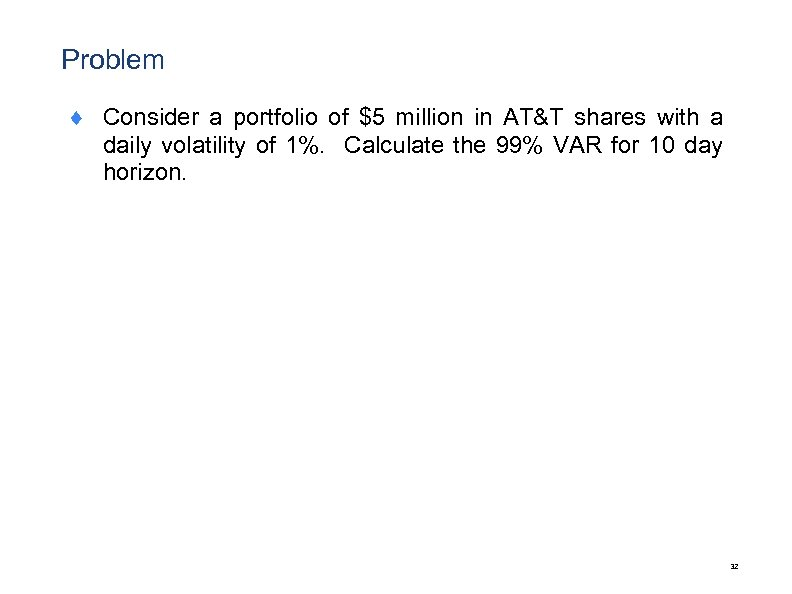Problem ¨ Consider a portfolio of \$5 million in AT&T shares with a daily volatility of 1%. Calculate the 99% VAR for 10 day horizon. 32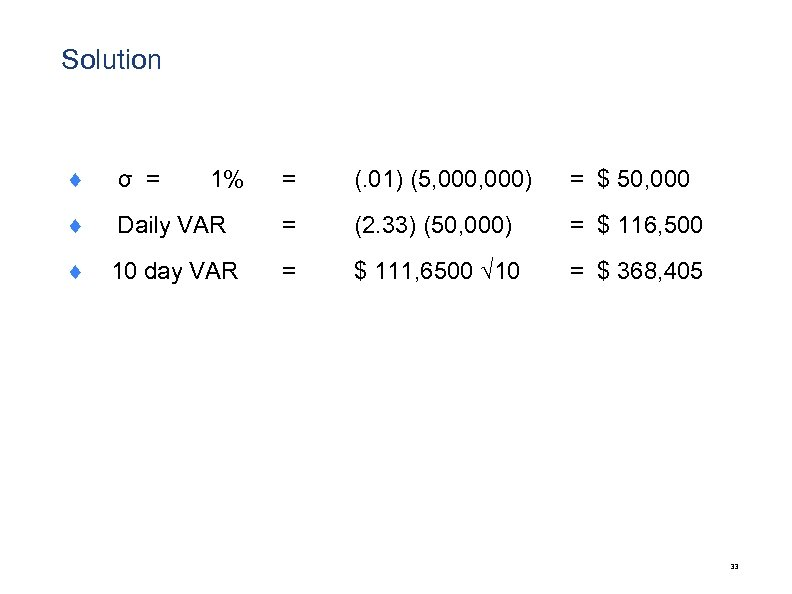Solution ¨ σ = 1% = (. 01) (5, 000) = \$ 50, 000 ¨ Daily VAR = (2. 33) (50, 000) = \$ 116, 500 ¨ 10 day VAR = \$ 111, 6500 √ 10 = \$ 368, 405 33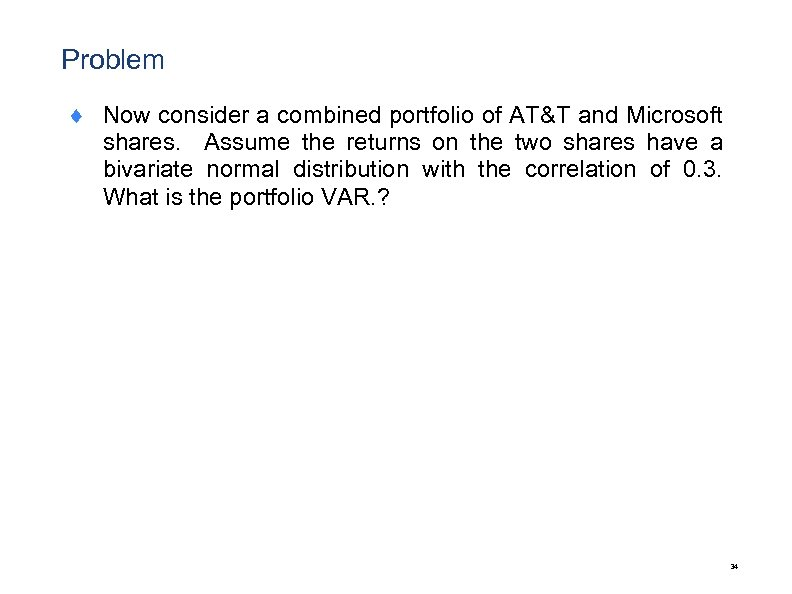Problem ¨ Now consider a combined portfolio of AT&T and Microsoft shares. Assume the returns on the two shares have a bivariate normal distribution with the correlation of 0. 3. What is the portfolio VAR. ? 34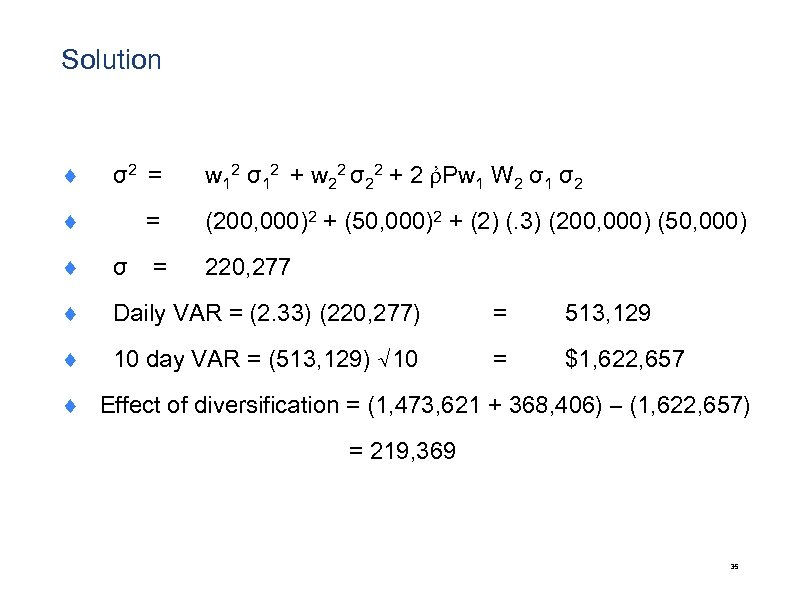Solution ¨ σ2 = w 12 σ12 + w 22 σ22 + 2 ῤPw 1 W 2 σ1 σ2 ¨ (200, 000)2 + (50, 000)2 + (2) (. 3) (200, 000) (50, 000) = ¨ σ = 220, 277 ¨ Daily VAR = (2. 33) (220, 277) = 513, 129 ¨ 10 day VAR = (513, 129) √ 10 = \$1, 622, 657 ¨ Effect of diversification = (1, 473, 621 + 368, 406) – (1, 622, 657) = 219, 369 35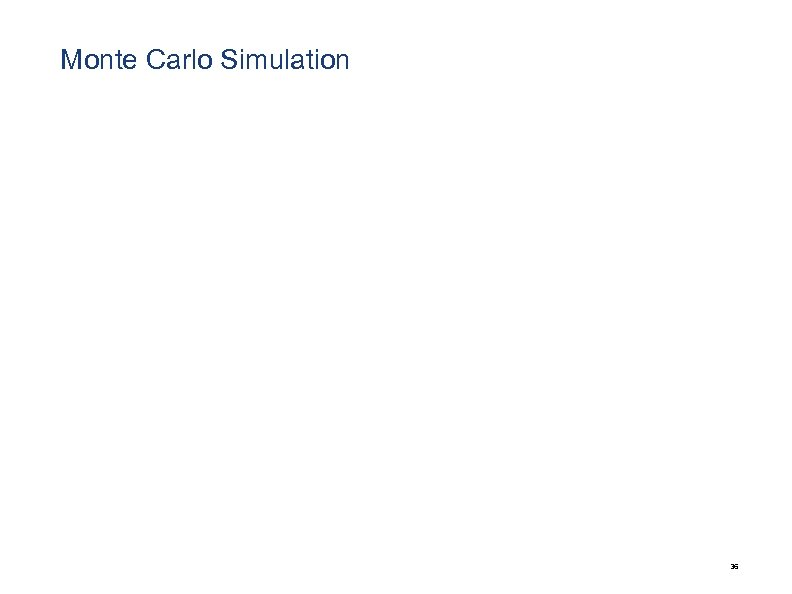Monte Carlo Simulation 36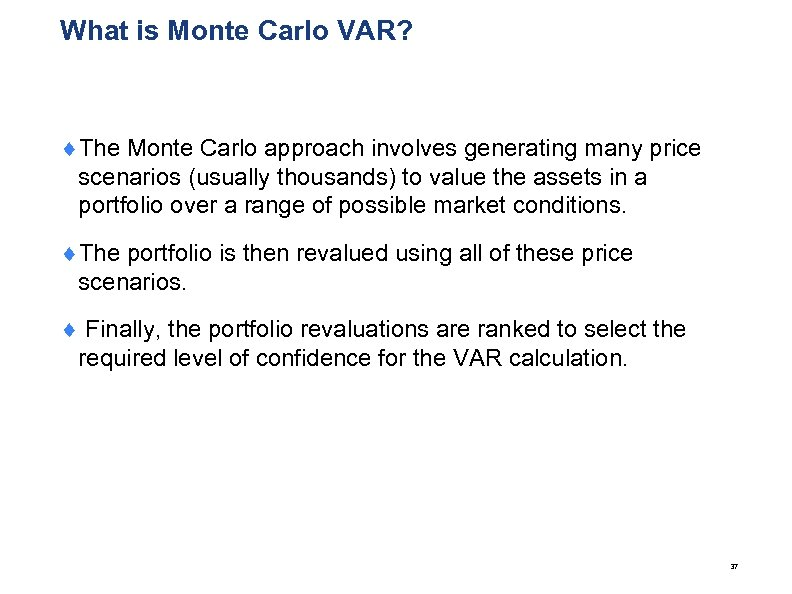What is Monte Carlo VAR? ¨The Monte Carlo approach involves generating many price scenarios (usually thousands) to value the assets in a portfolio over a range of possible market conditions. ¨The portfolio is then revalued using all of these price scenarios. ¨ Finally, the portfolio revaluations are ranked to select the required level of confidence for the VAR calculation. 37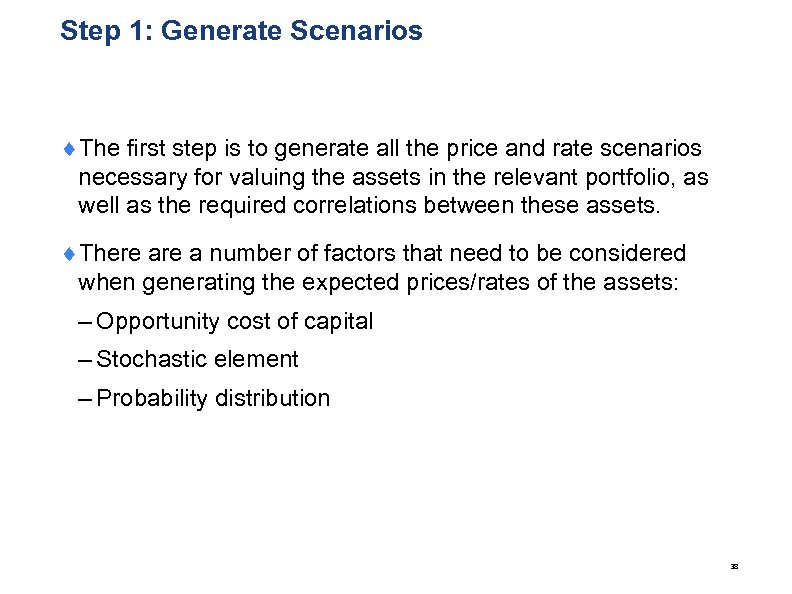Step 1: Generate Scenarios ¨The first step is to generate all the price and rate scenarios necessary for valuing the assets in the relevant portfolio, as well as the required correlations between these assets. ¨There a number of factors that need to be considered when generating the expected prices/rates of the assets: – Opportunity cost of capital – Stochastic element – Probability distribution 38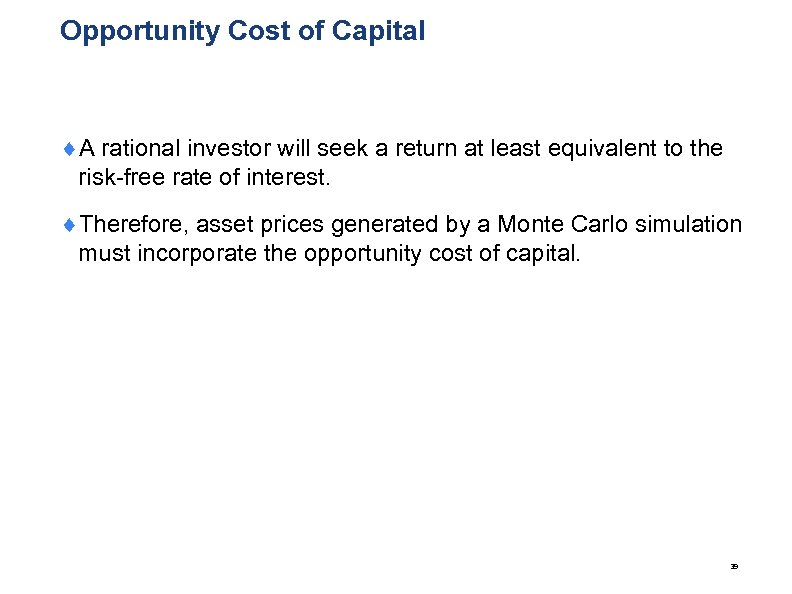Opportunity Cost of Capital ¨A rational investor will seek a return at least equivalent to the risk-free rate of interest. ¨Therefore, asset prices generated by a Monte Carlo simulation must incorporate the opportunity cost of capital. 39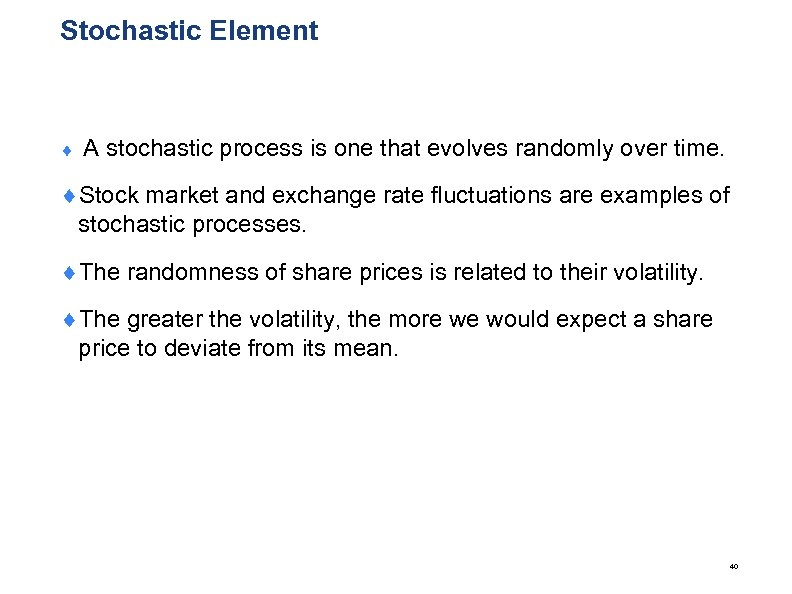Stochastic Element ¨ A stochastic process is one that evolves randomly over time. ¨Stock market and exchange rate fluctuations are examples of stochastic processes. ¨The randomness of share prices is related to their volatility. ¨The greater the volatility, the more we would expect a share price to deviate from its mean. 40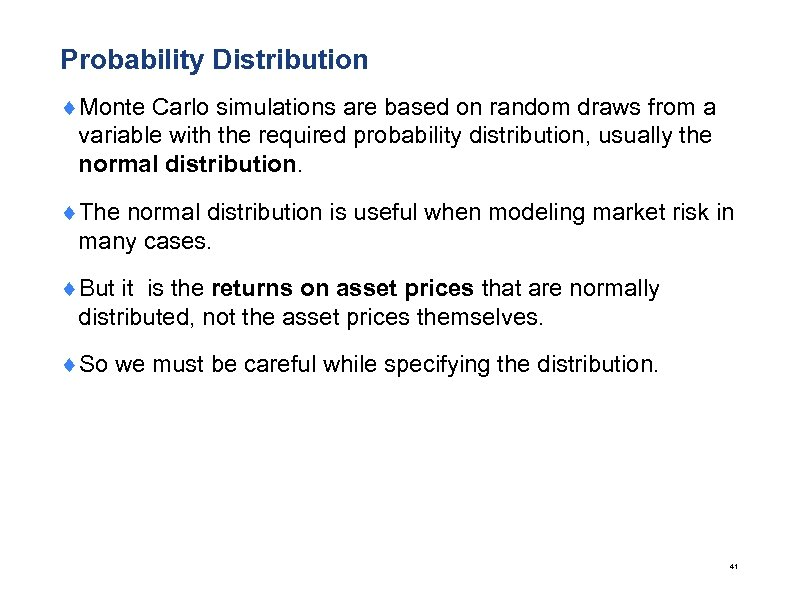Probability Distribution ¨Monte Carlo simulations are based on random draws from a variable with the required probability distribution, usually the normal distribution. ¨The normal distribution is useful when modeling market risk in many cases. ¨But it is the returns on asset prices that are normally distributed, not the asset prices themselves. ¨So we must be careful while specifying the distribution. 41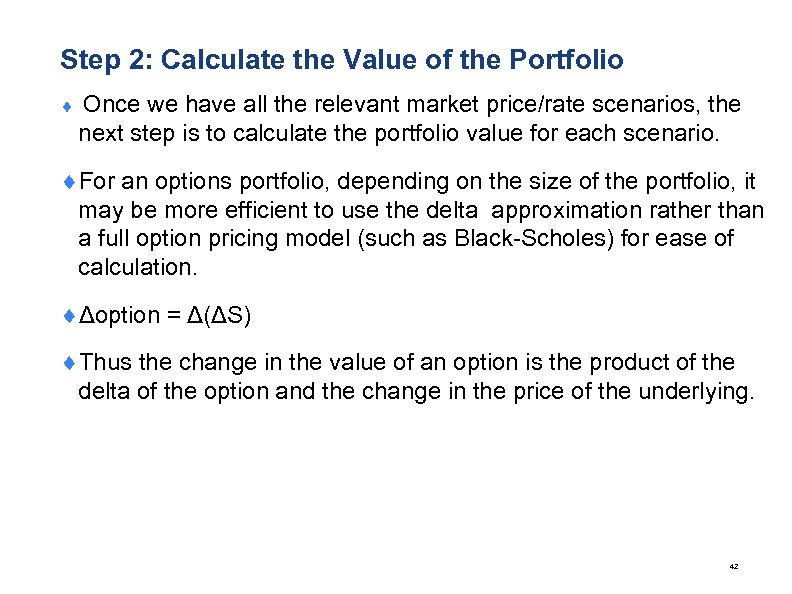Step 2: Calculate the Value of the Portfolio ¨ Once we have all the relevant market price/rate scenarios, the next step is to calculate the portfolio value for each scenario. ¨For an options portfolio, depending on the size of the portfolio, it may be more efficient to use the delta approximation rather than a full option pricing model (such as Black-Scholes) for ease of calculation. ¨Δoption = Δ(ΔS) ¨Thus the change in the value of an option is the product of the delta of the option and the change in the price of the underlying. 42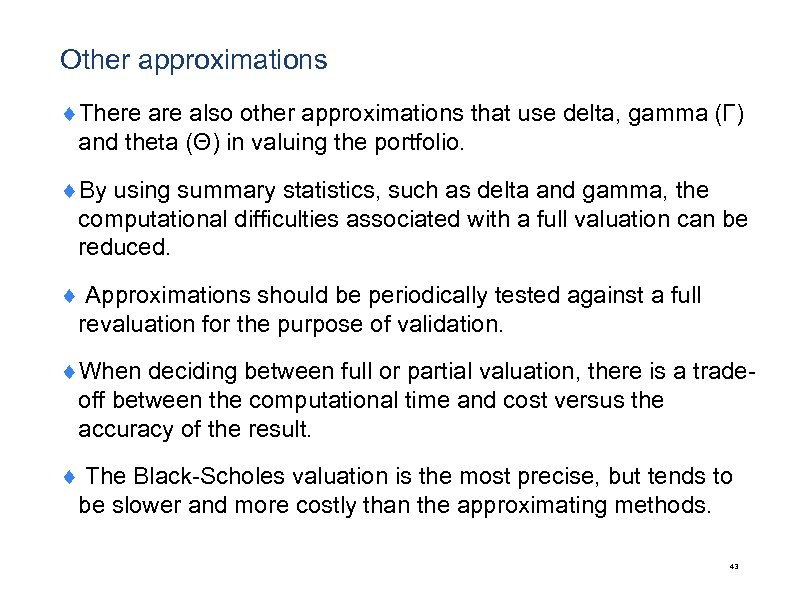Other approximations ¨There also other approximations that use delta, gamma (Γ) and theta (Θ) in valuing the portfolio. ¨By using summary statistics, such as delta and gamma, the computational difficulties associated with a full valuation can be reduced. ¨ Approximations should be periodically tested against a full revaluation for the purpose of validation. ¨When deciding between full or partial valuation, there is a tradeoff between the computational time and cost versus the accuracy of the result. ¨ The Black-Scholes valuation is the most precise, but tends to be slower and more costly than the approximating methods. 43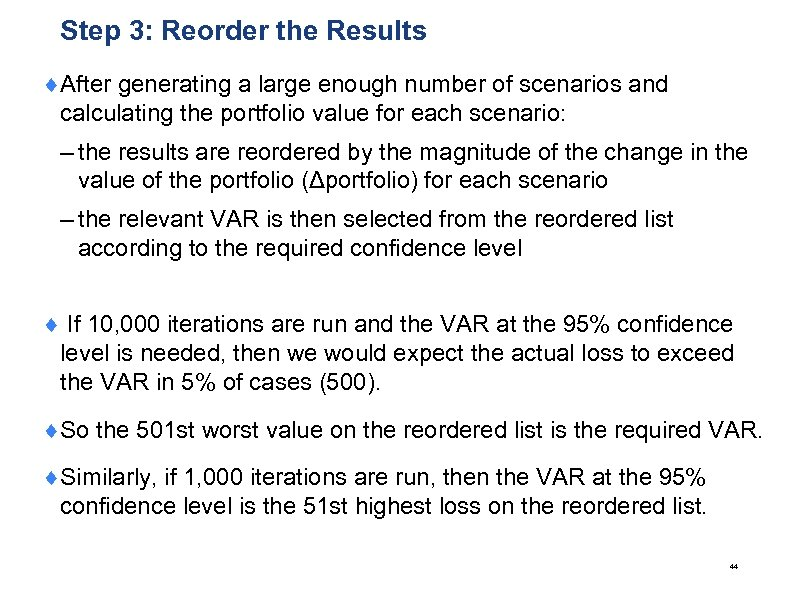Step 3: Reorder the Results ¨After generating a large enough number of scenarios and calculating the portfolio value for each scenario: – the results are reordered by the magnitude of the change in the value of the portfolio (Δportfolio) for each scenario – the relevant VAR is then selected from the reordered list according to the required confidence level ¨ If 10, 000 iterations are run and the VAR at the 95% confidence level is needed, then we would expect the actual loss to exceed the VAR in 5% of cases (500). ¨So the 501 st worst value on the reordered list is the required VAR. ¨Similarly, if 1, 000 iterations are run, then the VAR at the 95% confidence level is the 51 st highest loss on the reordered list. 44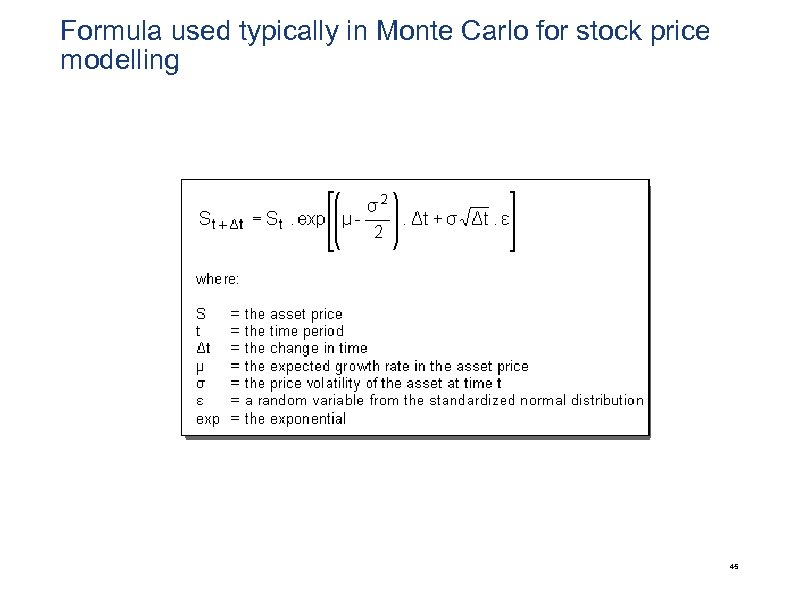Formula used typically in Monte Carlo for stock price modelling 45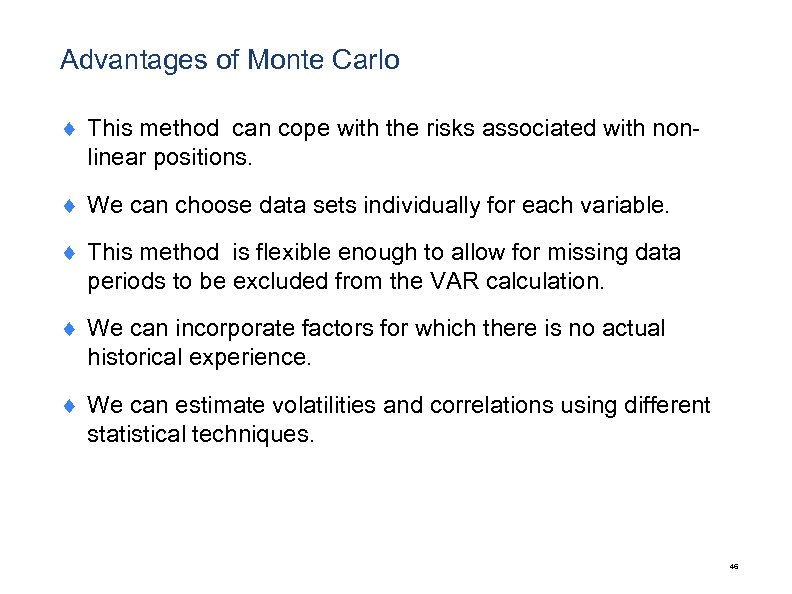Advantages of Monte Carlo ¨ This method can cope with the risks associated with nonlinear positions. ¨ We can choose data sets individually for each variable. ¨ This method is flexible enough to allow for missing data periods to be excluded from the VAR calculation. ¨ We can incorporate factors for which there is no actual historical experience. ¨ We can estimate volatilities and correlations using different statistical techniques. 46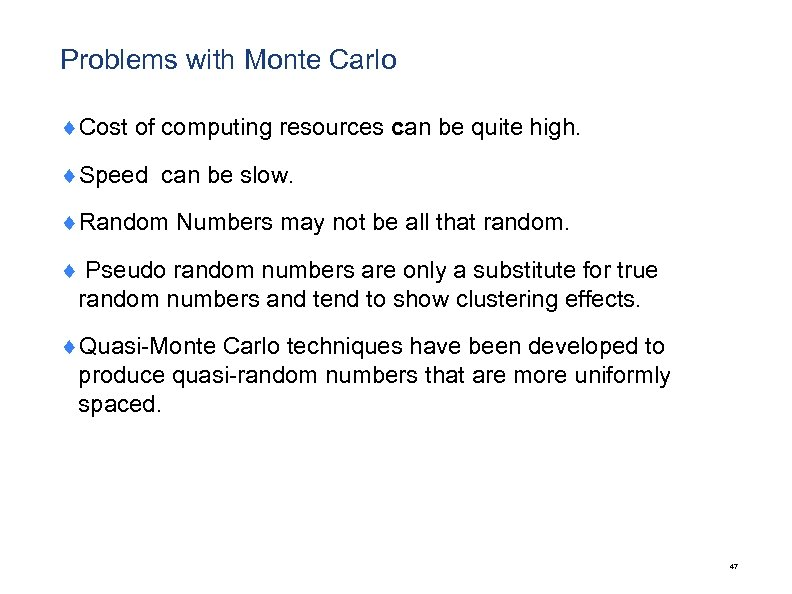Problems with Monte Carlo ¨Cost of computing resources can be quite high. ¨Speed can be slow. ¨Random Numbers may not be all that random. ¨ Pseudo random numbers are only a substitute for true random numbers and tend to show clustering effects. ¨Quasi-Monte Carlo techniques have been developed to produce quasi-random numbers that are more uniformly spaced. 47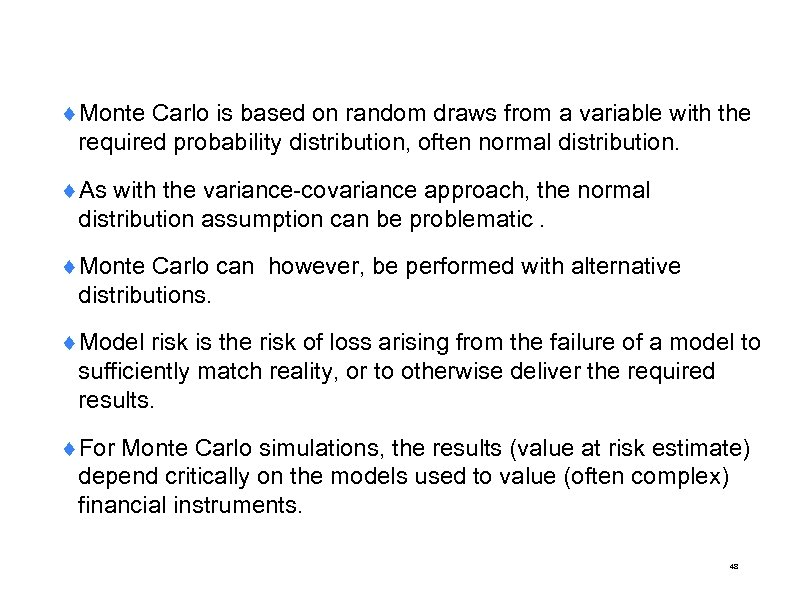¨Monte Carlo is based on random draws from a variable with the required probability distribution, often normal distribution. ¨As with the variance-covariance approach, the normal distribution assumption can be problematic. ¨Monte Carlo can however, be performed with alternative distributions. ¨Model risk is the risk of loss arising from the failure of a model to sufficiently match reality, or to otherwise deliver the required results. ¨For Monte Carlo simulations, the results (value at risk estimate) depend critically on the models used to value (often complex) financial instruments. 48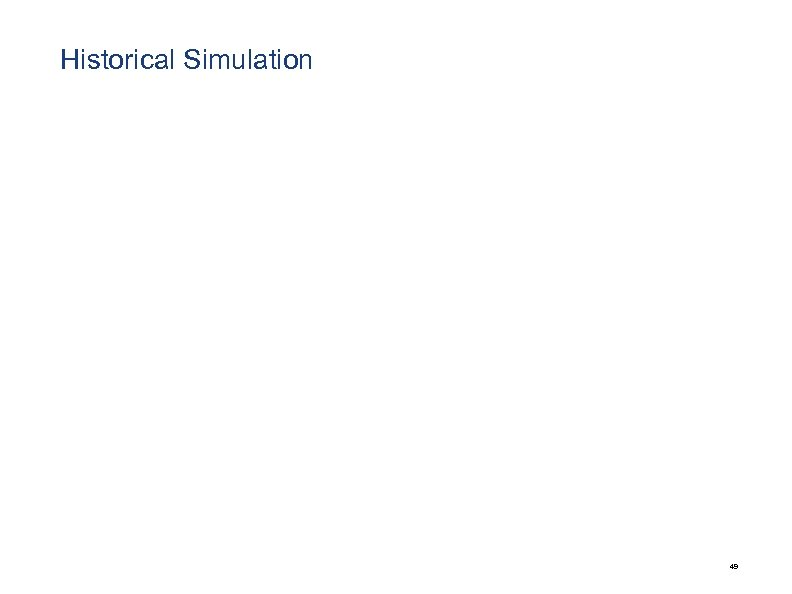Historical Simulation 49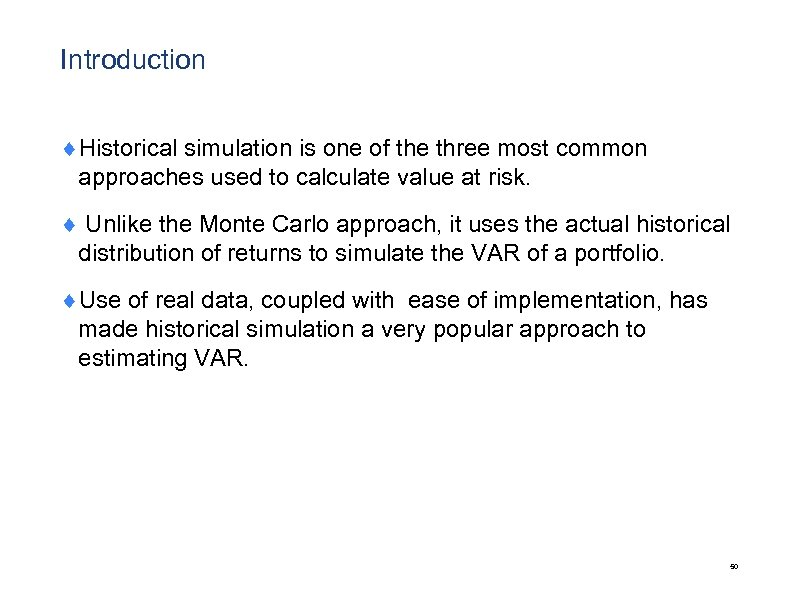Introduction ¨Historical simulation is one of the three most common approaches used to calculate value at risk. ¨ Unlike the Monte Carlo approach, it uses the actual historical distribution of returns to simulate the VAR of a portfolio. ¨Use of real data, coupled with ease of implementation, has made historical simulation a very popular approach to estimating VAR. 50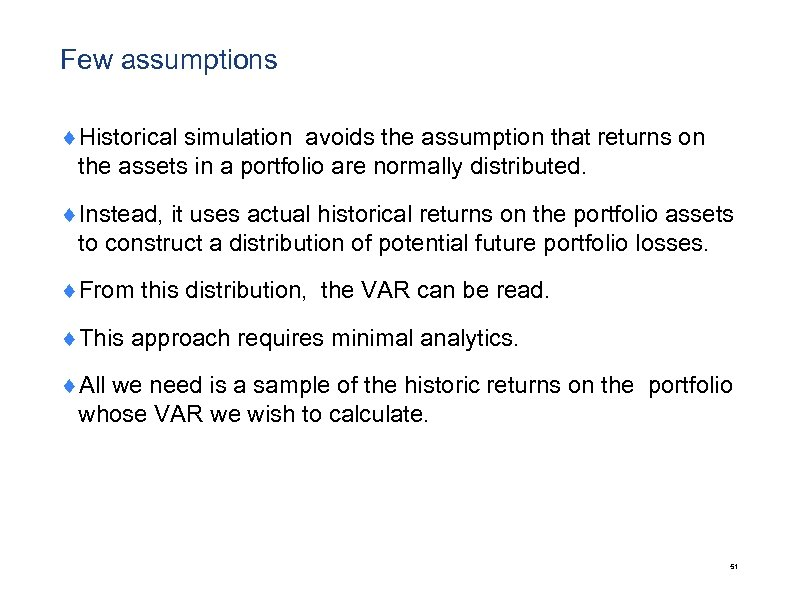Few assumptions ¨Historical simulation avoids the assumption that returns on the assets in a portfolio are normally distributed. ¨Instead, it uses actual historical returns on the portfolio assets to construct a distribution of potential future portfolio losses. ¨From this distribution, the VAR can be read. ¨This approach requires minimal analytics. ¨All we need is a sample of the historic returns on the portfolio whose VAR we wish to calculate. 51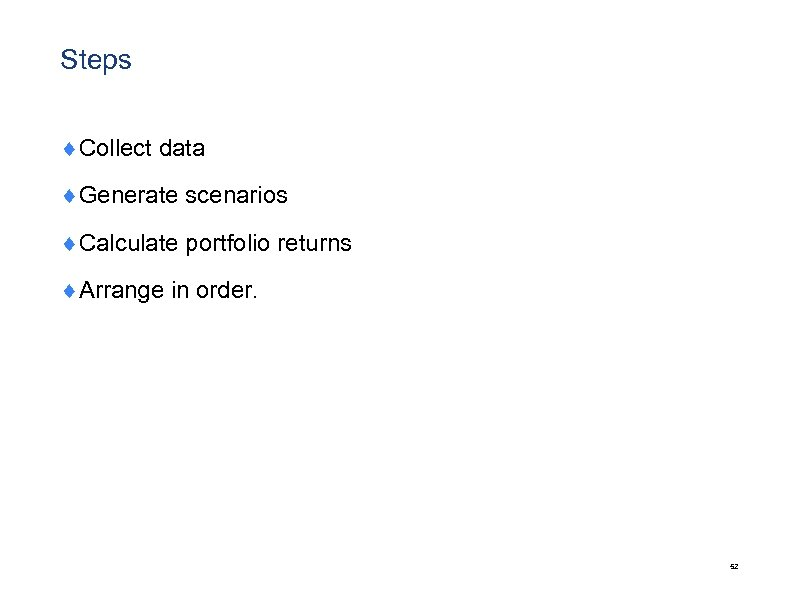Steps ¨Collect data ¨Generate scenarios ¨Calculate portfolio returns ¨Arrange in order. 52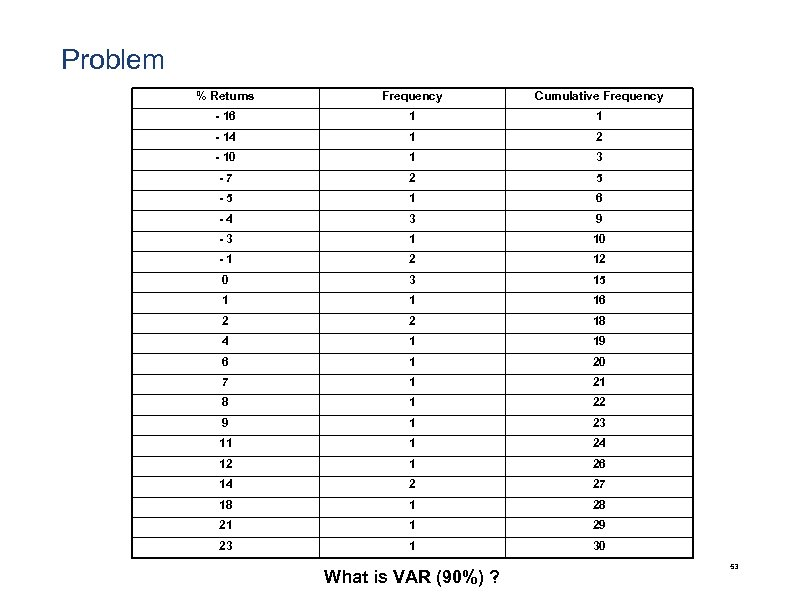Problem % Returns Frequency Cumulative Frequency - 16 1 1 - 14 1 2 - 10 1 3 -7 2 5 -5 1 6 -4 3 9 -3 1 10 -1 2 12 0 3 15 1 1 16 2 2 18 4 1 19 6 1 20 7 1 21 8 1 22 9 1 23 11 1 24 12 1 26 14 2 27 18 1 28 21 1 29 23 1 30 What is VAR (90%) ? 53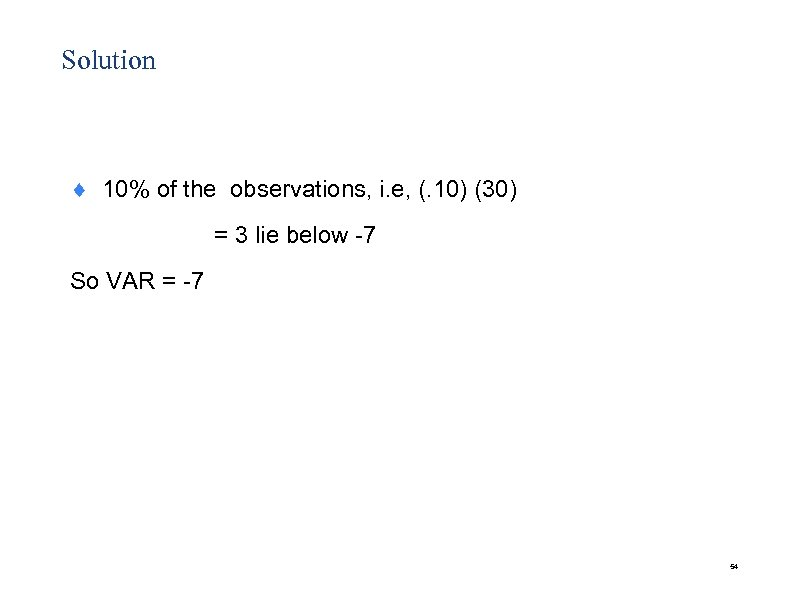Solution ¨ 10% of the observations, i. e, (. 10) (30) = 3 lie below -7 So VAR = -7 54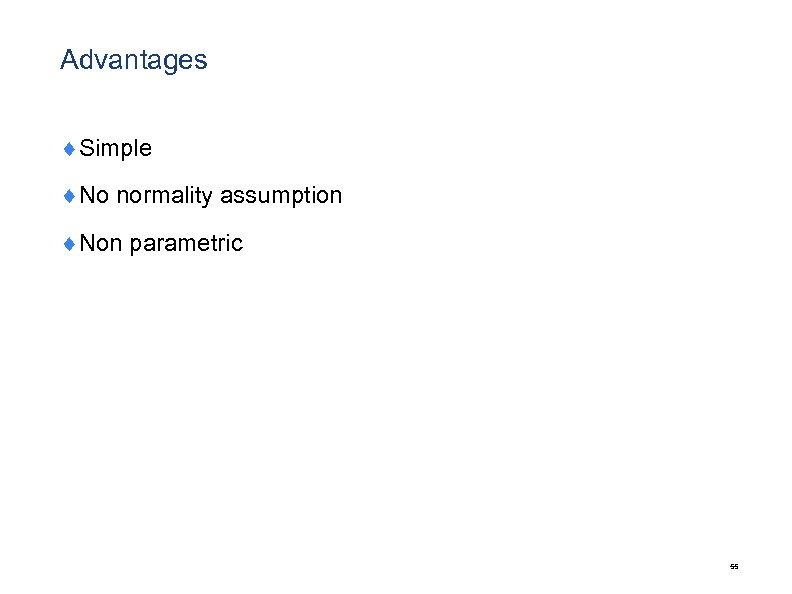Advantages ¨Simple ¨No normality assumption ¨Non parametric 55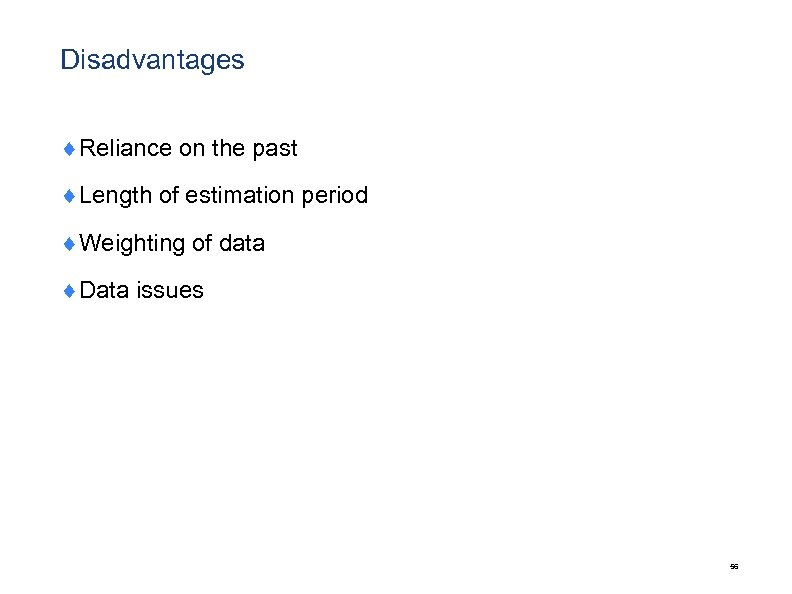Disadvantages ¨Reliance on the past ¨Length of estimation period ¨Weighting of data ¨Data issues 56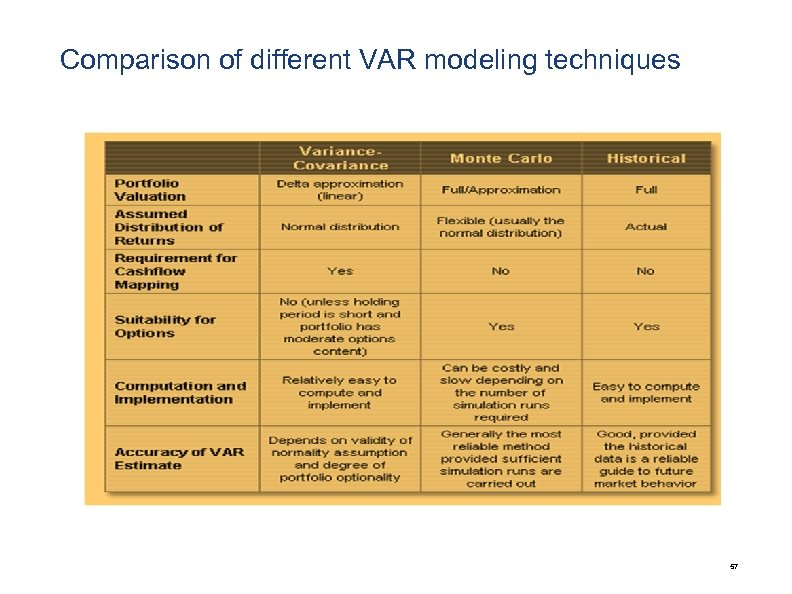Comparison of different VAR modeling techniques 57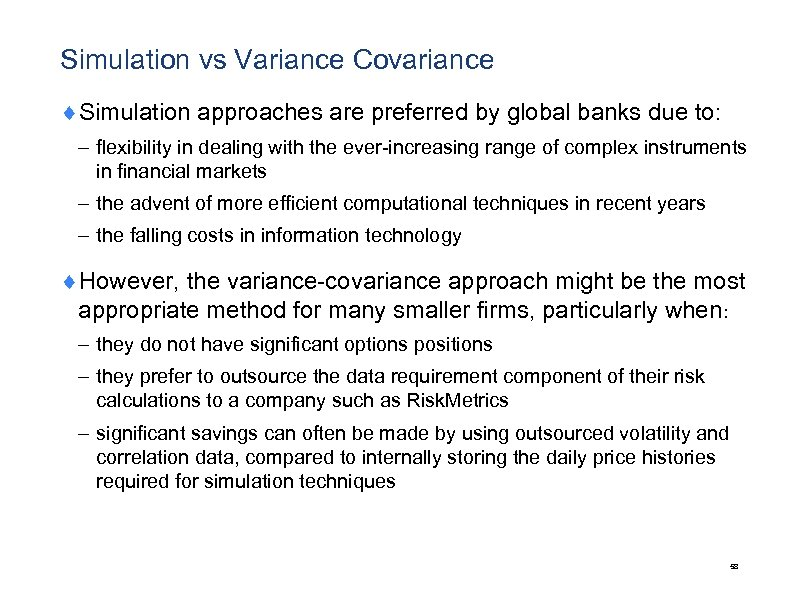Simulation vs Variance Covariance ¨Simulation approaches are preferred by global banks due to: – flexibility in dealing with the ever-increasing range of complex instruments in financial markets – the advent of more efficient computational techniques in recent years – the falling costs in information technology ¨However, the variance-covariance approach might be the most appropriate method for many smaller firms, particularly when: – they do not have significant options positions – they prefer to outsource the data requirement component of their risk calculations to a company such as Risk. Metrics – significant savings can often be made by using outsourced volatility and correlation data, compared to internally storing the daily price histories required for simulation techniques 58Model Validation 59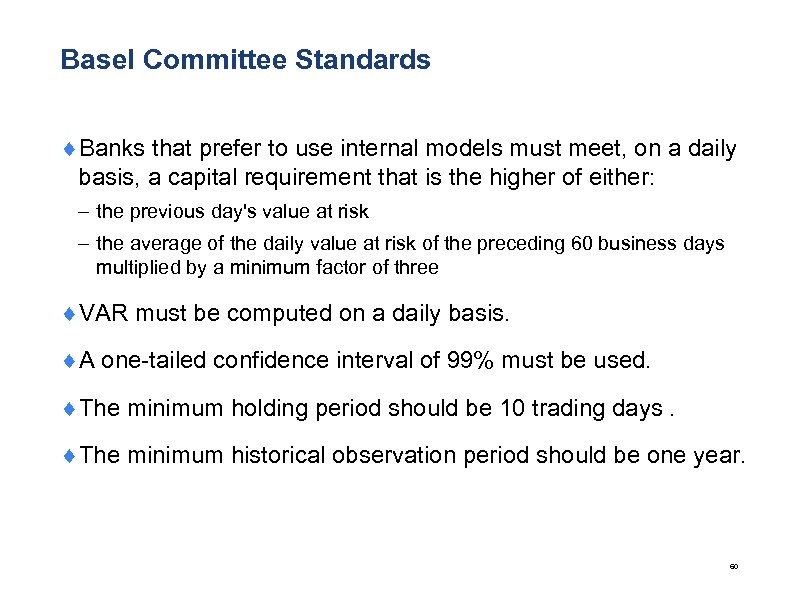Basel Committee Standards ¨Banks that prefer to use internal models must meet, on a daily basis, a capital requirement that is the higher of either: – the previous day's value at risk – the average of the daily value at risk of the preceding 60 business days multiplied by a minimum factor of three ¨VAR must be computed on a daily basis. ¨A one-tailed confidence interval of 99% must be used. ¨The minimum holding period should be 10 trading days. ¨The minimum historical observation period should be one year. 60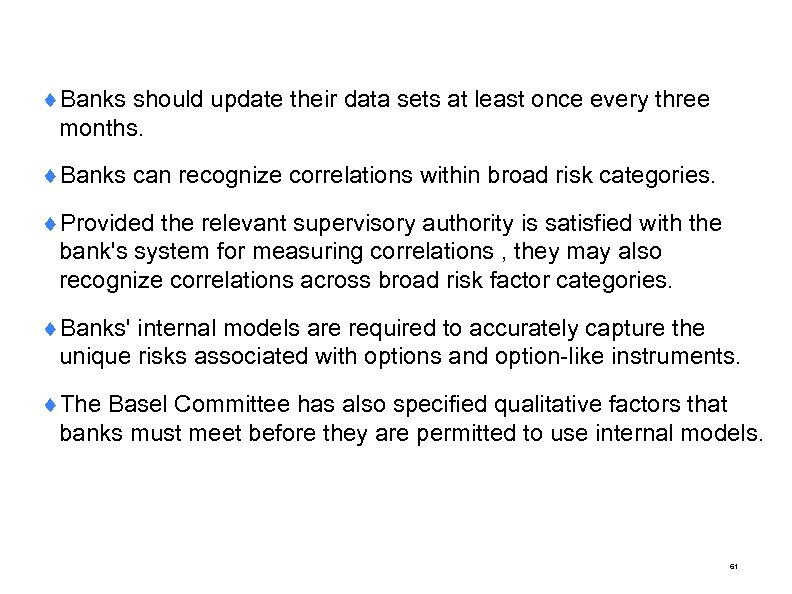¨Banks should update their data sets at least once every three months. ¨Banks can recognize correlations within broad risk categories. ¨Provided the relevant supervisory authority is satisfied with the bank's system for measuring correlations , they may also recognize correlations across broad risk factor categories. ¨Banks' internal models are required to accurately capture the unique risks associated with options and option-like instruments. ¨The Basel Committee has also specified qualitative factors that banks must meet before they are permitted to use internal models. 61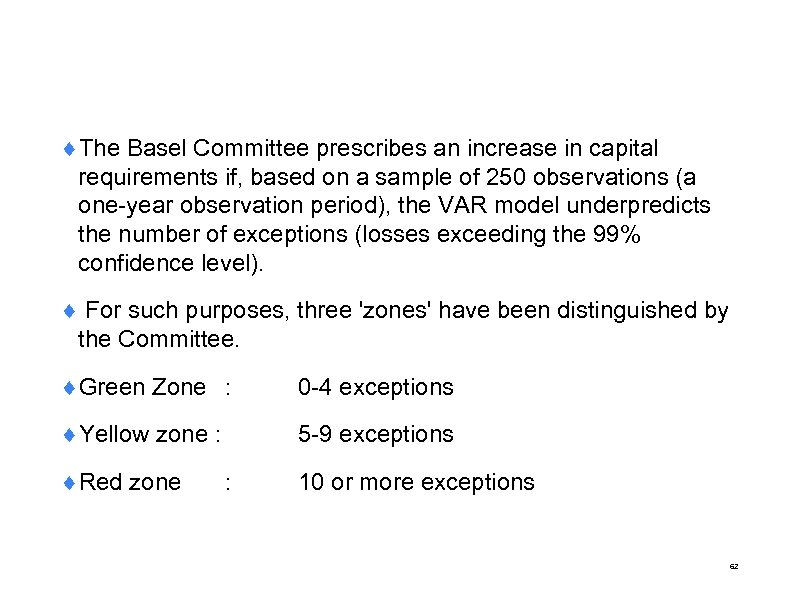¨The Basel Committee prescribes an increase in capital requirements if, based on a sample of 250 observations (a one-year observation period), the VAR model underpredicts the number of exceptions (losses exceeding the 99% confidence level). ¨ For such purposes, three 'zones' have been distinguished by the Committee. ¨Green Zone : 0 -4 exceptions ¨Yellow zone : 5 -9 exceptions ¨Red zone 10 or more exceptions : 62Stress Testing 63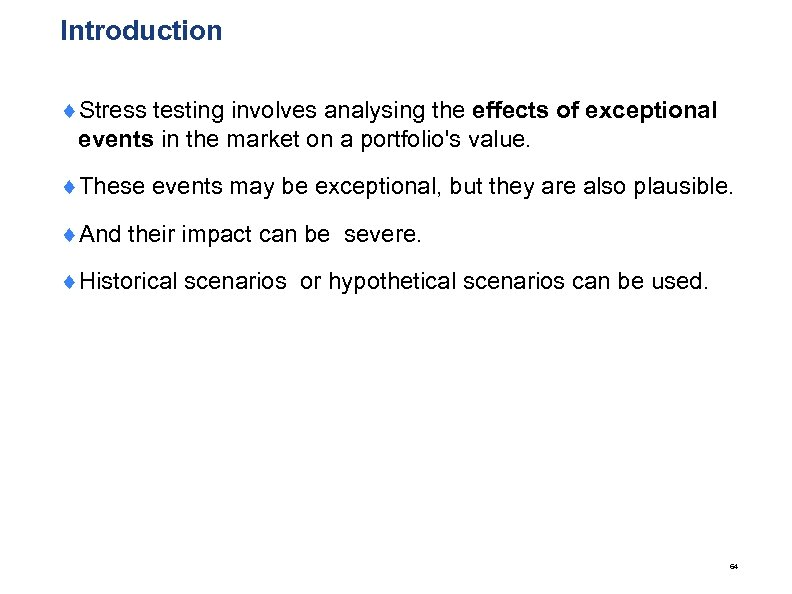Introduction ¨Stress testing involves analysing the effects of exceptional events in the market on a portfolio's value. ¨These events may be exceptional, but they are also plausible. ¨And their impact can be severe. ¨Historical scenarios or hypothetical scenarios can be used. 64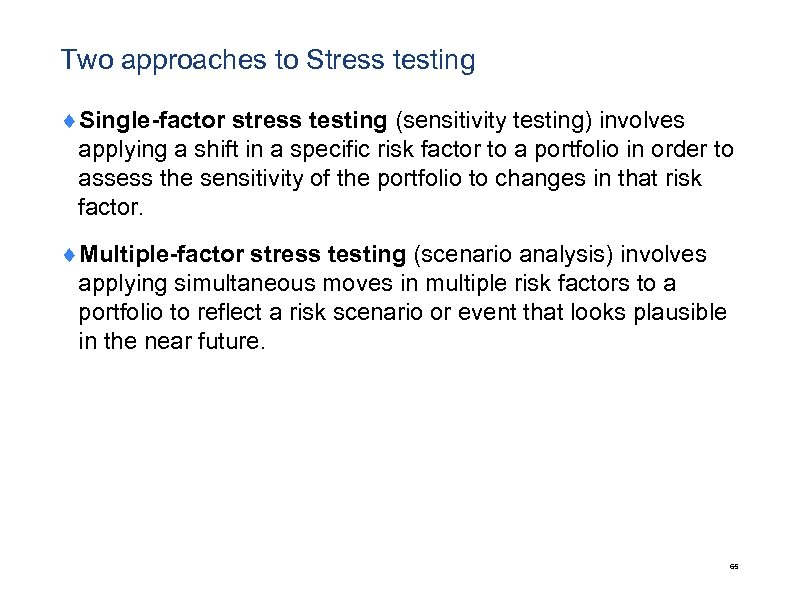Two approaches to Stress testing ¨Single-factor stress testing (sensitivity testing) involves applying a shift in a specific risk factor to a portfolio in order to assess the sensitivity of the portfolio to changes in that risk factor. ¨Multiple-factor stress testing (scenario analysis) involves applying simultaneous moves in multiple risk factors to a portfolio to reflect a risk scenario or event that looks plausible in the near future. 65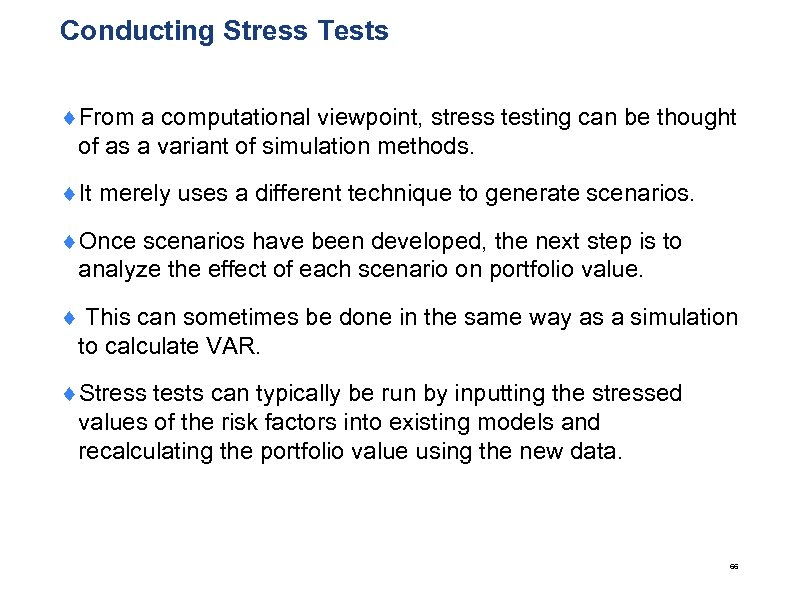Conducting Stress Tests ¨From a computational viewpoint, stress testing can be thought of as a variant of simulation methods. ¨It merely uses a different technique to generate scenarios. ¨Once scenarios have been developed, the next step is to analyze the effect of each scenario on portfolio value. ¨ This can sometimes be done in the same way as a simulation to calculate VAR. ¨Stress tests can typically be run by inputting the stressed values of the risk factors into existing models and recalculating the portfolio value using the new data. 66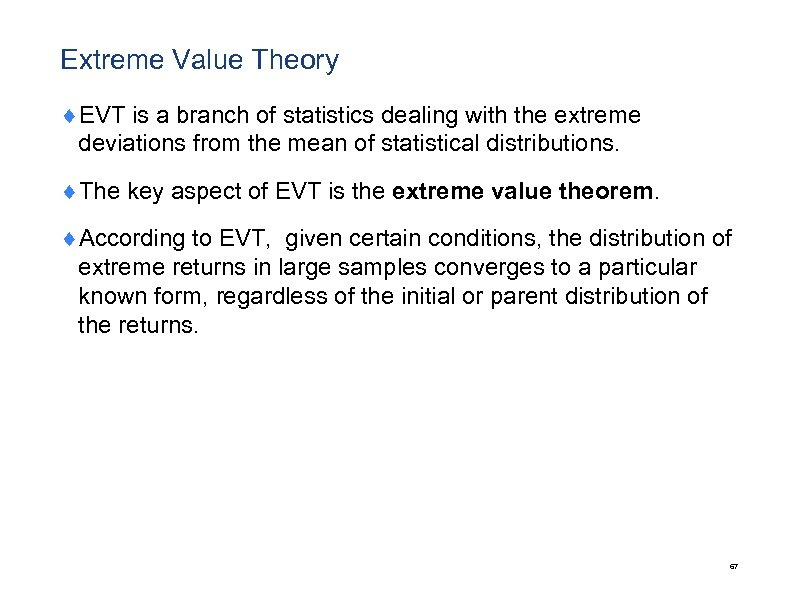Extreme Value Theory ¨EVT is a branch of statistics dealing with the extreme deviations from the mean of statistical distributions. ¨The key aspect of EVT is the extreme value theorem. ¨According to EVT, given certain conditions, the distribution of extreme returns in large samples converges to a particular known form, regardless of the initial or parent distribution of the returns. 67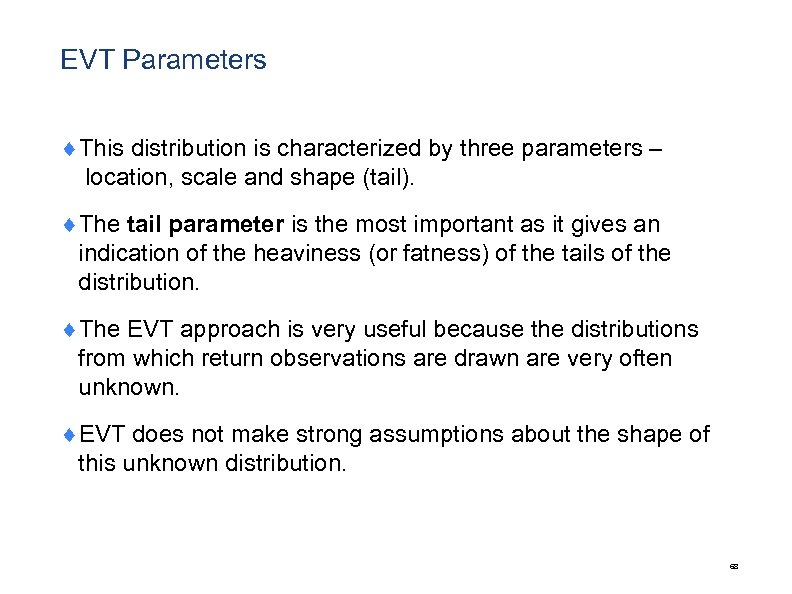EVT Parameters ¨This distribution is characterized by three parameters – location, scale and shape (tail). ¨The tail parameter is the most important as it gives an indication of the heaviness (or fatness) of the tails of the distribution. ¨The EVT approach is very useful because the distributions from which return observations are drawn are very often unknown. ¨EVT does not make strong assumptions about the shape of this unknown distribution. 68